• and左右两个表达式为True，即返回结果为True； B选项中， 在逻辑运算符中，其优先级not>and>or，即not True为False， False or False返回结果False； C选项中， not True返回结果False，F...
正确答案： A,D 你的答案： B
参考解析：

本题考察的是逻辑运算符优先级的使用。
A选项中，1<2返回结果 True，
and左右两个表达式都为True，即返回结果为True；
B选项中，
在逻辑运算符中，其优先级为not>and>or，即not True为False，
False or False返回结果为False；
C选项中，
not True返回结果为False，False and True返回结果为False；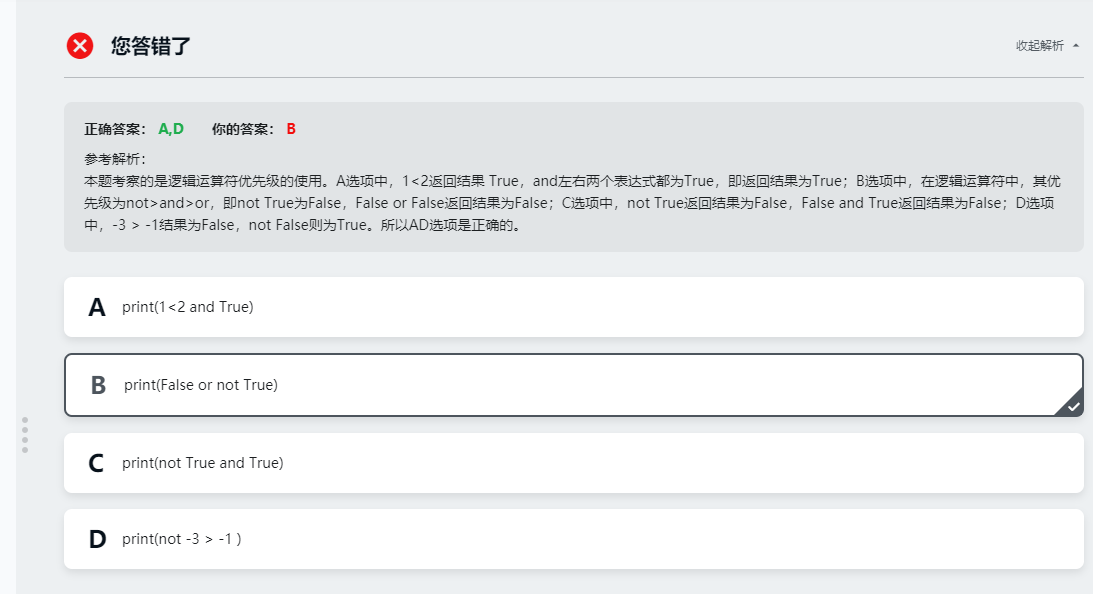展开全文练习
• ## Java正则表达式

千次阅读 2019-07-06 19:03:50
java.util.regex程序包只包含用于实现Java正则表达式处理技术的两个类，分别名Pattern和Matcher。自然而然你会想到正则表达式由模式匹配（pattern matching）而成。java.lang还定义了一个新接口，它支持这些新的类...
正则表达式基础
参考书籍：精通正则表达式。
Java正则表达式API
java.util.regex程序包只包含用于实现Java正则表达式处理技术的两个类，分别名为Pattern和Matcher。自然而然你会想到正则表达式由模式匹配（pattern matching）而成。java.lang还定义了一个新接口，它支持这些新的类。在研究Patternt和Matcher之前，我们先快速浏览一下CharSequence这一新概念。另外，为方便起见String类为运行正则表达式匹配提供了一些新程序作为捷径
CharSequence接口
正则表达式是根据字符序列进行模式匹配的。虽然String对象封装了字符序列，但是它们并不是能够这样做的唯一对象。
JDK 1.4定义了一个名为CharSequence的新接口，可描述特定不变的字符序列。该新接口是一个抽象(abstraction)，它把字符序列从包含这些字符的具体实现(specific implementation)中分离出来。JDK 1.4对“年高德勋”的String和StringBuffer类进行了改进，用于实现CharSequence接口。新的CharBuffer类也实现了CharSequence。CharSequence接口也在字符集映射中投入了使用
CharSequence定义的API十分简单。毕竟它没有花太多“笔墨”描述字符序列。
package java.lang;
public interface CharSequence
{
int length();
char charAt (int index);
public String toString();
CharSequence subSequence (int start, int end);
}
CharSequence描述的每个字符序列通过length( )方法会返回某个长度值。通过调用charAt( )可以得到序列的各个字符，其中索引是期望的字符位置（desired character position）。字符位置从零到字符序列的长度之间，与我们熟悉的String.charAt( )基本一样。
toString( )方法返回的String对象包括所描述的字符序列。这可能很有用，如打印字符序列。正如之前提过的，String现在实现了CharSequence。String和CharSequence同为不变的，因此如果CharSequence描述一个完整的String，那么CharSequence的toString( )方法返回的是潜在的String对象而不是副本。如果备份对象是StringBuffer或CharBuffer，系统将创建一个新的String保存字符序列的副本。
最后通过调用subSequence( )方法会创建一个新的CharSequence描述子范围（subrange）。start和end的指定方式与String.substring( )的方式相同：start必须是序列的有效索引（valid index）；end必须比start大，标志的是最末字符的索引加一。换句话说，start是起始索引（计算在内），end是结束索引（不计算在内）。
CharSequence接口因为没有赋值方法（mutator method）看上去似乎是不变的，但是基本的实现对象可能不是不变的。CharSequence方法反映了基本对象的现状。如果状态改变，CharSequence方法返回的信息同样会发生变化。如何你依赖CharSequence保持稳定且不确认基础的实现，你可以调用toString( )方法，对字符序列拍个真实不变的快照。
Pattern类
Pattern类封装了正则表达式，它是你希望在目标字符序列中检索的模式。匹配正则表达式的代价可能非常高昂，因为可能排列数量巨大，尤其是模式反复应用的情况。大部分正则表达式处理器（包括Perl在内，在封装中）首先会编译表达式，然后利用编译好的表达式在输入中进行模式检测。
在这一点上Java正则表达式程序包别无两样。Pattern类的实例是将一个编译好的正则表达式封装起来。让我们看看完整的Pattern API，看看它是如何使用的。记住，这并不是一个句法完整的类文件，它只中去掉了类主体的方法签名。
package java.util.regex;
public final class Pattern implements java.io.Serializable
{
public static final int UNIX_LINES
public static final int CASE_INSENSITIVE
public static final int MULTILINE
public static final int DOTALL
public static final int UNICODE_CASE
public static final int CANON_EQ
public static boolean matches (String regex, CharSequence input)
public static Pattern compile (String regex)
public static Pattern compile (String regex, int flags)
public String pattern()
public int flags()
public String[] split (CharSequence input, int limit)
public String[] split (CharSequence input)
public Matcher matcher (CharSequence input)
}
上面所列的第一个方法matches( )是个公用程序。它可以进行完整的匹配操作，并根据正则表达式是否匹配整个的（entire）输入序列返回一个布尔值。这种方法很容易上手，因为你无须追踪任何对象；你要做的仅是调用一个简单的静态方法并测试结果。

这种方法适用于默认设置尚可接受并且只需进行一次测试的情况。假如你要重复检查同一模式，假如你要找的模式是输入的子序列，又假如你要设置非默认选项，那么你应当创建一个新的Pattern对象并使用新对象的API方法。
需要注意的是Pattern类并没有public 构造函数。只有通过调用静态工厂方法才可以创建新的实例。compile( )的两个形式采用的都是正则表达式的String参数。返回的Pattern对象包含被转换成已编译内部形式的正则表达式。如果你提供的正则表达式形态异常，那么compile( )工厂方法会抛出java.util.regex.PatternSyntaxException（模式句法异常）。这是未经检查的异常，因此如果你对自己使用的正则表达式是否可行存在疑虑（例如它传递给你是一个变量），那么你可以把对compile( )的调用放到try/catch块中进行检测。
compile( )的第二种形式接受标志有一个位掩码,这影响了正则表达式的默认编译。这些标志启用了可选的编译模式行为，例如如何处理边界或不区分大小写等。（除CANOB_EQ外）这些标志（flag）同样可由嵌入表达式内的子表达式启用。标志可以与布尔或（OR）表达式结合使用，如下所示：

Pattern pattern = Pattern.compile ("[A-Z][a-zA-Z]*", Pattern.CASE_INSENSITIVE | Pattern.UNIX_LINES);

Pattern类的实例是不变的，各个实例与对应的正则表达式绑定，无法修改。Pattern对象也是线程安全的，可被多个线程同时使用。
Matcher类
Matcher类为匹配字符序列的正则表达式模式提供了丰富的API。Matcher实例常常通过对Pattern对象调用matcher( )方法来创建的，它常常采用由该Pattern封装的正则表达式：
Matcher类的实例是监控状态型对象，它们封装了与特定输入字符序列匹配的具体正则表达式。Matcher对象并不是线程安全的，因为它们在方法调用之间有保有内状态（hold internal state）。一个Matcher实例来自一个Pattern实例，Matcher对象的pattern( )返回的是向后引用（back reference），指向创建了Matcher的Pattern对象。Matcher对象可以重复使用，但是因其监控状态属性，为了开始新匹配操作它们必须处于已知状态。这可通过调用reset( )方法来实现，该方法在与匹配程序有关的CharSequence之前为模式匹配备好了对象。无参数的reset( )将使用上次为Matcher设置的CharSequence。如果你希望对新的字符序列进行匹配，那么你可以将一个新的CharSequence传递给reset( )，随后匹配将针对目标进行。例如，随着你读取各行的文件，你可以把它传递给reset( )。
matches( )，如果整个（entire）字符序列匹配正则表达式的模式，则它返回true。反之如果模式匹配的只是子序列，方法将返回false。在文件中，这种方法用于选取恰好满足一定模式的行是非常有用的。这种行为（behavior）与作用于Pattern类的公用程序matches( )相同。
lookingAt( )方法与matches( )相似，但是它不要求整个序列的模式匹配。如果正则表达式模式匹配字符序列的beginning（开头），则lookingAt( )返回true。lookingAt( )方法往往从序列的头部开始扫描。该方法的名字暗示了匹配程序正在“查看”目标是否以模式开头。如果返回为true，那么可以调用start( )、end( )和group( )方法匹配的子序列的范围（随后将给出更多关于这些程序的内容）。
find( )方法运行的是与lookingAt( )相同类型的匹配操作，但是它会记住前一个匹配的位置并在之后重新开始扫描。从而允许了相继调用find( )对输入进行逐句比对，寻找嵌入的匹配。复位后第一次调用该方法，则扫描将从输入序列的首个字符开始。在随后调用中，它将从前一个匹配的子序列后面的第一个字符重新开始扫描。如各个调用来说，如果找到了模式将返回true；反之将返回false。通常你会使用find( )循环访问一些文本来查找其中所有匹配的模式。
带位置参数的find( )会在给定的索引位置进行隐式复位并从该位置开始扫描。然后如果需要可以调用无参数的find( )扫描输入序列剩余的部分。
一旦检查到匹配，你可以通过调用start( )和end( )确定匹配位于字符序列的什么位置。Start( )方法返回的是匹配序列首个字符的索引；end( )方法返回的值等于匹配序列最末字符的索引加一。这些返回值与CharSequence.subsequence( )的返回值一致，可直接用于提取匹配的子序列。

CharSequence subseq;
if (matcher.find( ))
{
subseq = input.subSequence (matcher.start(), matcher.end( ));
}
一些正则表达式可以匹配空字符串，这种情况下start( )和end( )将返回相同的值。只有当匹配之前已经过matches( )、lookingAt( )或检测find( )的检测，start( )和end( )返回的值才有意义。如果没有检测到匹配或最后的匹配尝试返回的是false，那么调用start( )或end( )将导致java.lang.IllegalStateException（Java语言非法状态异常）。为了了解带有group参数的start( )和end( )，我们首先需要知道表达式捕获组（expression capture group）。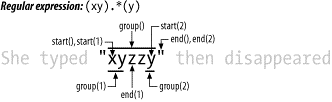正则表达式可能包含称为捕获组（capture group）的子表达式，它们被小括号括了起来。在正则表达式的求值期间将保存匹配这些捕获组表达式的输入子序列。一旦完全匹配操作完成，这些保存的代码片断可通过确定相应的组号从Matcher对象上重新获取。捕获组可以嵌套使用，数量可以通过从左到右计算左括弧（开括号）得到。无论整个表达式是否有子组，它的捕获组总能记为组零（group zero）。例如，正则表达式A((B)(C(D)))可能有的捕获组编号如表5-3所示。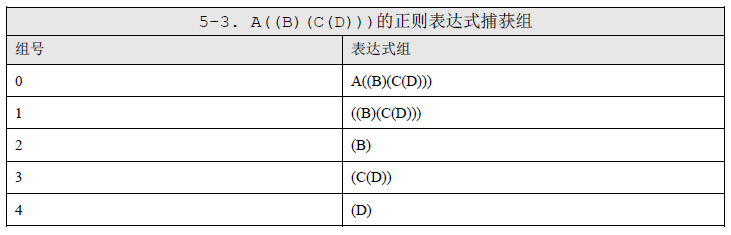这种分组句法存在异常事件。以(?开头的组是个纯的（pure）或说是无法捕获的组。它的值无法保存且它对无法计算捕获组编号。
捕获组在正则表达式模式中的编号由groupCount( )方法返回。该值来自原始的Pattern对象，是不可变的。组号必须为正且小于groupCount( )返回的值。传递超出范围的组号将导致java.lang.IndexOutOfBoundsException（java语言索引出界异常）。
可以将捕获组号传递给start( )和end( )来确定子序列是否匹配已知的捕获组子表达式。有可能出现这样一种情况，即整个表达式成功匹配但是有一个或多个的捕获组无法匹配。如果请求的捕获组当前没有设置则start( )和end( )方法的返回值将为-1。
（正如之前看到的）你可以利用start( )和end( )返回的值从输入的CharSequence中提取出匹配的子序列，但是group( )方法为此提供了更简单的方式。调用带数字参数的group( )将返回一个字段，该字段是匹配特殊捕获组的子序列。如果你调用的group( )不含参数，则返回将是与整个正则表达式（组零）匹配的子序列。

String match0 = input.subSequence (matcher.start(), matcher.end()).toString( ); String match2 = input.subSequence (matcher.start (2), matcher.end (2)).toString( ); 上述代码与下列代码等效： String match0 = matcher.group( );  String match2 = matcher.group(2);

最后让我们看看Matcher对象解决修改字符序列的方法。正则表达式最常见的应用之一是查找并替换（search-and-replace）。这种应用使用replaceFirst( )和replaceAll( )可以轻轻松松就搞定。它们的行为方式是相同的，区别在于replaceFirst( )在找到第一个匹配后就会停止，而replaceAll( )将循环执行直到替换完所有的匹配。二者都带有String参数，String参数是用于替换输入字符序列中匹配模式的替换值（replacement value）
上文提过，捕获组在正则表达式内可以向后引用（back-reference）。它们也可以被你提供组replaceFirst( )或replaceAll( )的替换字符串引用。捕获组号通过添加美元符号$可嵌入替换字符串中。当替换字符串被替换成结果字符串时，每次出现的$g将被group( )返回的值代替。如果你想在替换字符串使用字面量（literal）美元符号，那么你必须在它前面加个反斜杠符号（\$）。如果想要传递反斜杠符号，你必须多加一个反斜杠（\\）。如果你想在捕获组引用后面跟上字面量的数值型数字，那么你可以用反斜杠将它们与组号分开，像这样：123$2\456。
Matcher对象记住的状态信息位（the bits of state information）之一是追加位置（append position）。追加位置是用于记住输入字符序列的量，这些字符序列已经通过之前调用appendReplacement( )复制了出来。当调用appendReplacement( )时，将发生如下过程： 1. 从输入中读取字符是从当前追加位置开始，读取的字符将被添加到已知的StringBuffer中。最后复制的字符就在匹配模式的首个字符之前。这个字符位于start( )返回的索引减一的位置。 2. 如先前描述的，替换字符串被添加给StringBuffer并替换任何嵌入的捕获组引用。 3. 追加位置更新成跟在匹配模式后面的字符的索引，这个索引是end( )返回的值。 仅当前一个匹配操作成功（通常调用find( )）appendReplacement( )方法才能正常工作。如果前一个匹配返回的是false或在复位后立即调用该方法，你将得到一个“令人愉快的奖励”：java.lang.IllegalStateException（java语言非法状态异常）。
String类的正则表达式方法

package java.lang;
public final class String
implements java.io.Serializable, Comparable, CharSequence
{
// This is a partial API listing
public boolean matches (String regex)
public String [] split (String regex)
public String [] split (String regex, int limit)
public String replaceFirst (String regex, String replacement)
public String replaceAll (String regex, String replacement)
}
正则表达式语句
参考：Java Pattern类的用法详解(正则表达式)

展开全文• \ 做转意，即通常在"\"后面的字符按原来意义解释，如/b/匹配字符"b"，当b前面加了反斜杆后/\b/，转意匹配一个单词的边界。 -或- 对正则表达式功能字符的还原，如"*"匹配它前面元字符0次或多次，/a*/将匹配a,...
• 下列Python语句的输出结果是 def f():pass print(type(f())) 结果： NoneType数据类型包含唯一None，主要用于表示空值，如没有返回值的函数的结果 上机实践：2~6 2. 编写程序，输入本金、年利率和年数，计算复利...
（还在更新中…） 这篇博客花费了我的大量时间和精力，从创作到维护；若认可本篇博客，希望给一个点赞、收藏
并且，遇到了什么问题，请在评论区留言，我会及时回复的

这本书对Python的知识点的描述很详细，而且排版看的很舒服

几个例题： 假装自己从零开始学，将一些有代表性、有意思的例题抽取出来部分复习题： 遇到有意思的复习题，我会拿出来，并且进行分析上机实践： 全部上机实践题的解题思路

文章目录
第一章 Python概述几个例题
第二章 Python语言基础选择题：1、3、7、8思考题：9上机实践：2~6案例研究：使用Pillow库处理图像文件
第三章 程序流程控制几个例题选择题：1、2、3填空题：6思考题：3~6上机实践：2~14案例研究：使用嵌套循环实现图像处理算法
第四章 常用内置数据类型几个例题选择题：11填空题：4、7、8、9、10、13、21思考题：5上机实践：2~14案例研究：科学计算和数据分析
第五章 序列数据类型几个例题选择题：4、5、7、11、12填空题：1、5、6、12、13、14思考题：2、3、5上机实践：2~6案例研究：猜单词游戏
第六章 输入和输出几个例题填空题：1、2例题及上机实践：2~5案例研究：21点扑克牌游戏
第七章 错误和异常处理几个例题案例研究：使用调试器调试Python程序
第八章 函数和函数式编程一些知识点总结和几个例题选择题：1~5思考题：4~11上机实践：2~5案例研究：井字棋游戏
第九章 面向对象的程序设计第十章 模块和客户端第十一章 算法与数据结构基础第十二章 图形用户界面案例研究：简易图形用户界面计算器
第十三章 图形绘制图形绘制模块：tkinter图形绘制模块：turtle
第十四章 数值日期和时间处理第十五章 字符串和文本处理第十六章 文件和数据交换第十七章 数据访问第十八章 网络编程和通信第十九章 并行计算：进程、线程和协程第二十章 系统管理

第一章 Python概述

几个例题
一：Python3.7.4下载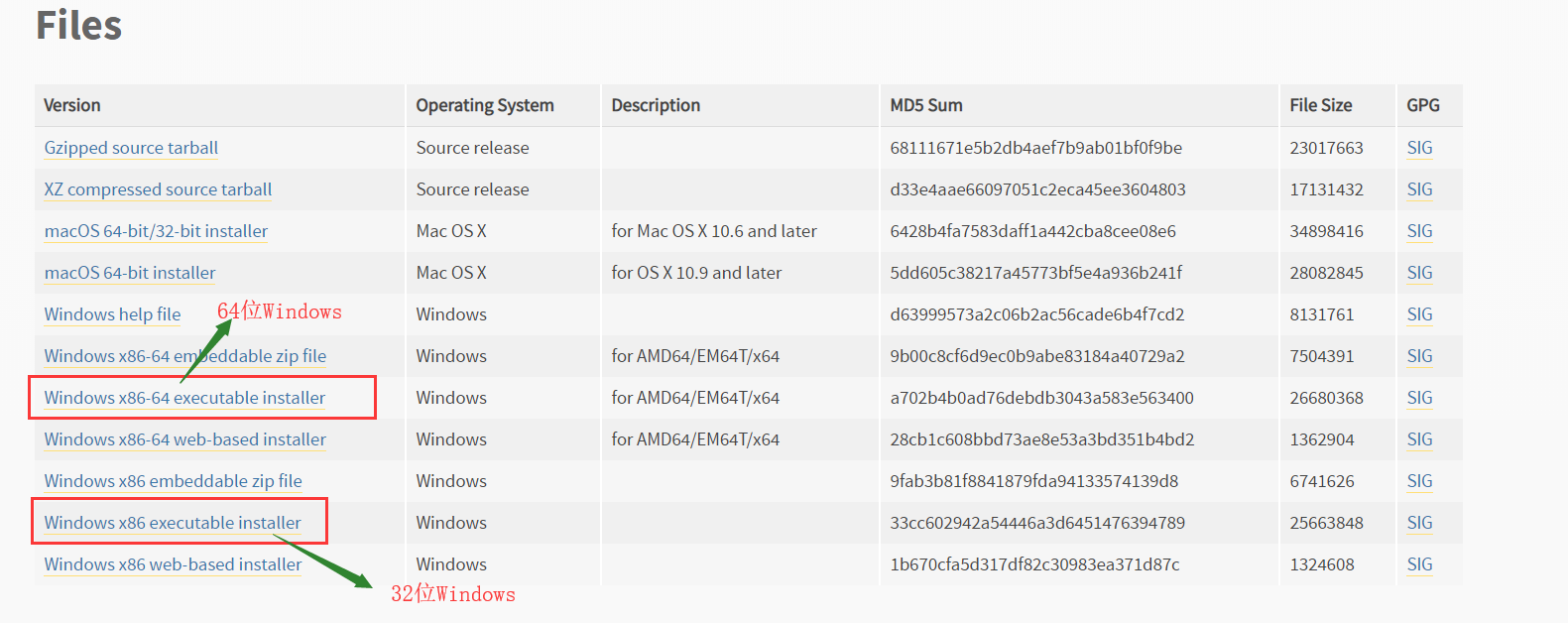下载，安装完python后：出现的四个玩意：Python 3.7 Module Docs，IDLE，Python 3.7 Manuals，Python 3.7（64-bit）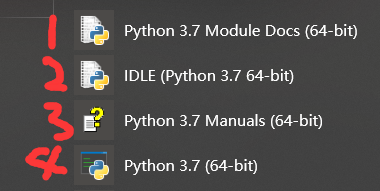Python 3.7 Module Docs（64-bit） 点击之后，会出现一个网页（将我下载的Python3.7.4文件夹中包含的模块都列了出来,页面不止这么点，还可以往下拉）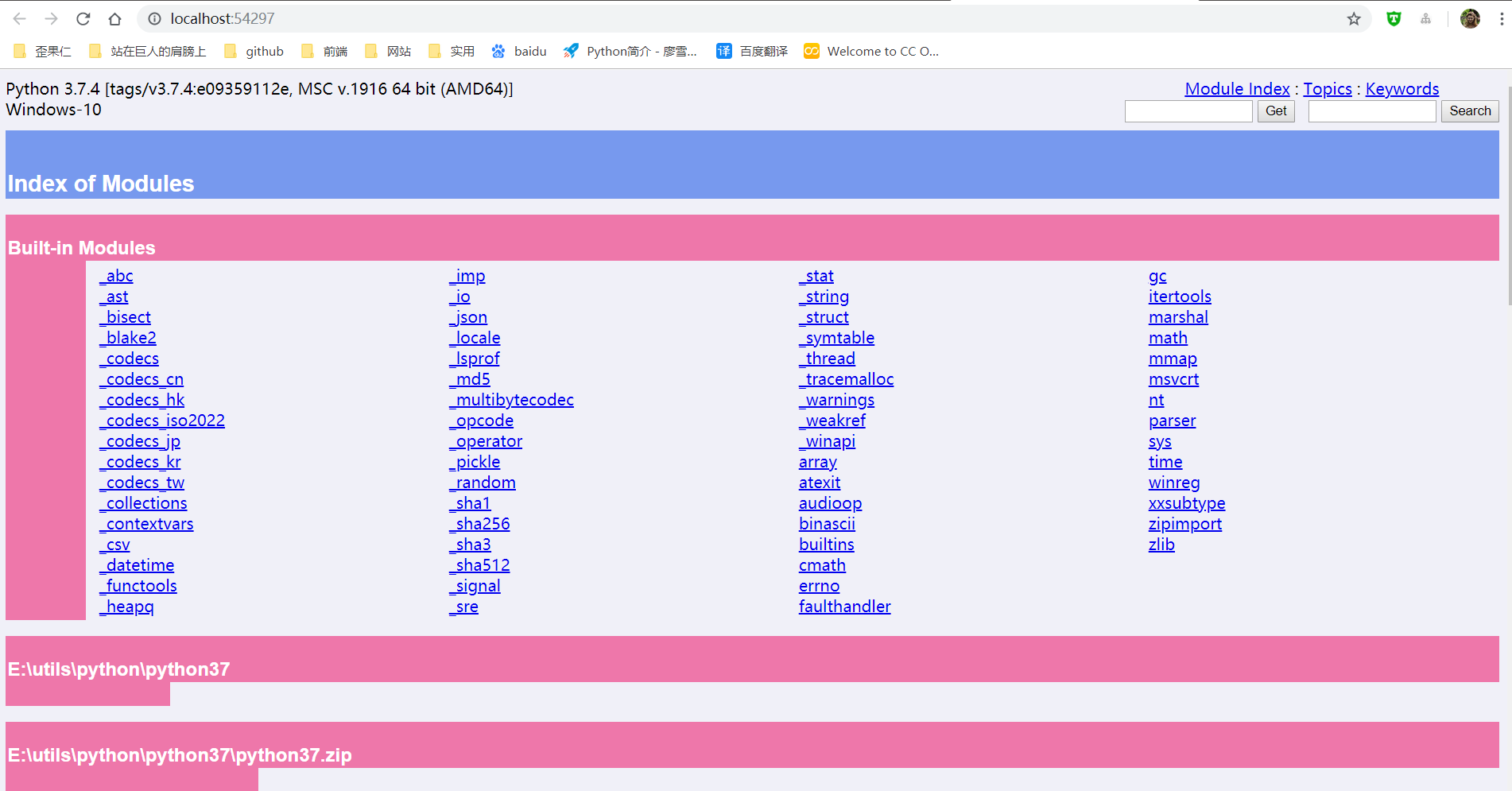IDLE（Python 3.7 64-bit） 一个Python编辑器，Python内置的集成开发工具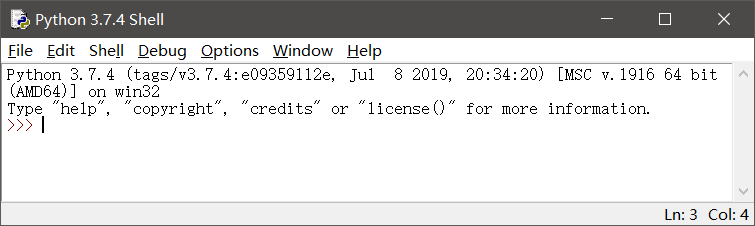Python 3.7 Manuals（64-bit） Python 3.7 开发手册  Python 3.7（64-bit） 控制台中运行Python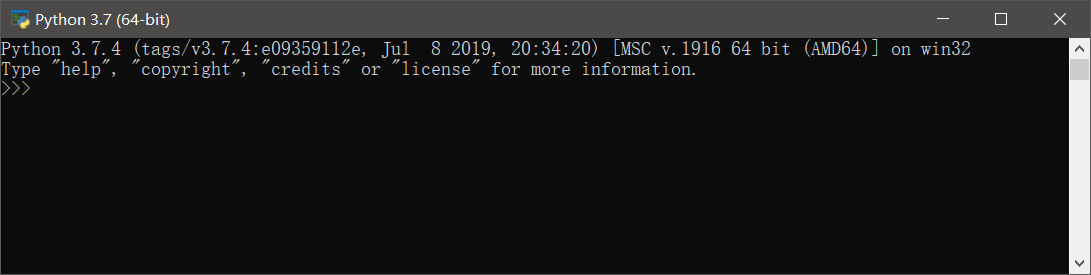二：更新pip和setuptools包，安装NumPy包，安装Matplotlib包
以下三个命令都是在控制台（windows中的cmd）中运行
更新pip和setuptools包
pip用于安装和管理Python扩展包setuptools用于发布Python包
python -m pip install -U pip setuptools

安装NumPy
Python扩展模块NumPy提供了数组和矩阵处理，以及傅立叶变换等高效的数值处理功能
 python -m pip install NumPy

安装Matplotlib包
Matplotlib是Python最著名的绘图库之一，提供了一整套和MATLAB相似的命令API，既适合交互式地进行制图，也可以作为绘图控件方便地嵌入到GUI应用程序中
python -m pip install Matplotlib

三：使用IDLE打开和执行Python源文件程序
首先： 有一个.py文件test.py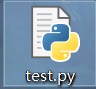使用IDLE打开.py文件的两种方式：
右键test.py---->Edit With IDLE---->Edit With IDLE 3.7（64-bit）打开IDLE，然后File---->Open（或者ctrl+O）选择.py文件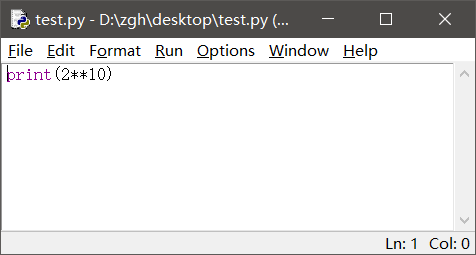运行
Run---->Run Module（或者F5） 就会出现这个界面，执行结果显示在这个界面中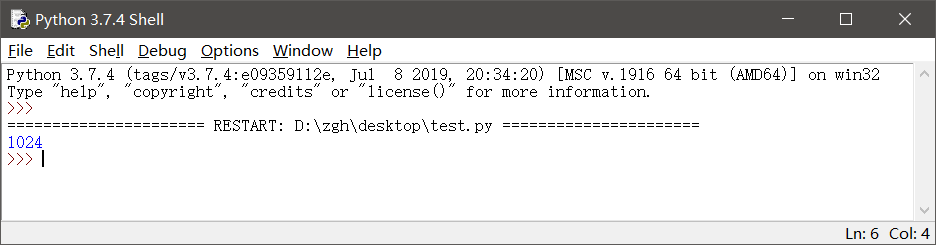补充一点： 如果在IDLE中编辑.py文件，记得修改后要保存（ctrl+s），再运行（F5）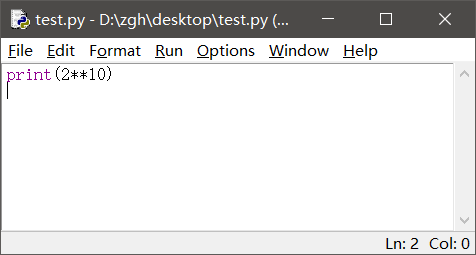四：使用资源管理器运行hello.py
hello.py文件在桌面
import random

print("hello,Python")
print("你今天的随机数字是：",random.choice(range(10)))#输出在0-9之间随机选择的整数
input()

在桌面打开PowerShell（还有两种输入方式：python hello.py或者.\hello.py）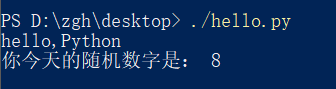或者在桌面打开cmd, 就输入hello.py或者python hello.py
补充：上述两种命令中的hello.py都是相对路径，因为文件在桌面，而且我是在桌面打开cmd，所以文件路劲可以这么简简单单的写。如果文件存储位置和cmd打开位置不一样，请使用绝对路径
五：命令行参数示例hello_argv.py
hello_argv.py文件在桌面
import sys

print("Hello,",sys.argv)
#这样写也行：
#print("Hello,"+sys.argv)

在桌面打开PowerShell（还有两种输入方式：python hello_argv.py 任意输入或者./hello_argv.py 任意输入）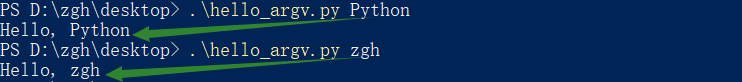或者在桌面打开cmd，就输入hello_argv.py 任意输入或者python hello_argv.py 任意输入
补充：以图中第一个命令举例，hello_argv.py即sys.argv；Python即sys.argv
第二章 Python语言基础

选择题：1、3、7、8
1. 在Python中，以下标识符合法的是
A. _B. 3CC. it’sB. str
答案：A

标识符的第一个字符必须是字母，下划线（_）；其后的字符可以是字母、下划线或数字。一些特殊的名称，作为python语言的保留关键字，不能作为标识符以双下划线开始和结束的名称通常具有特殊的含义。例如__init__为类的构造函数，一般应避免使用

B：以数字开头，错误 C：使用了'，不是字母、下划线或数字 D：str是保留关键字
3. 在下列Python语句中非法的是
A. x = y =1B. x = (y =1)C. x,y = y,xB. x=1;y=1
答案：B，C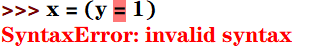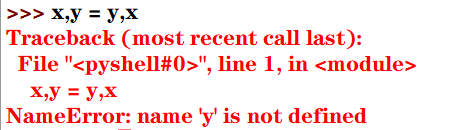7. 为了给整型变量x，y，z赋初值10，下面Python赋值语句正确的是
A. xyz=10B. x=10 y=10 z=10C. x=y=z=10B. x=10，y=10，z=10
答案：C

分号;用于在一行书写多个语句python支持链式赋值

A：赋值对象是xyz B：分号;用于在一行书写多个语句，而不是' '（即空格） D：分号;用于在一行书写多个语句，而不是,
8. 为了给整型变量x，y，z赋初值5，下面Python赋值语句正确的是
A. x=5;y=5;z=5B. xyz=5C. x,y,z=10B. x=10，y=10，z=10
答案：A

Pytho能支持序列解包赋值，但是变量的个数必须与序列的元素个数一致，否则会报错

B：赋值对象是xyz C：序列解包赋值，变量的个数必须与序列的元素个数一致，否则会报错 D：分号;用于在一行书写多个语句，而不是,
思考题：9
9.下列Python语句的输出结果是
def f():pass
print(type(f()))

结果：<class 'NoneType'>

NoneType数据类型包含唯一值None，主要用于表示空值，如没有返回值的函数的结果

上机实践：2~6
2. 编写程序，输入本金、年利率和年数，计算复利（结果保留两位小数）
money = int(input("请输入本金："))
rate = float(input("请输入年利率："))
years = int(input("请输入年数："))
amount = money*((1+rate/100)**years)
print(str.format("本金利率和为：{0:2.2f}",amount))

运行：
请输入本金：1000
请输入年利率：6.6
请输入年数：10
本金利率和为：1894.84

3. 编写程序，输入球的半径，计算球的表面积和体积（结果保留两位小数）
import math
r = float(input("请输入球的半径："))
area = 4 * math.pi * r**2
volume = 4/3*math.pi*r**3
print(str.format("球的表面积为：{0:2.2f}，体积为：{1:2.2f}",area,volume))

运行：
请输入球的半径：666
球的表面积为：5573889.08，体积为：1237403376.70

4. 编写程序，声明函数getValue(b,r,n)，根据本金b，年利率r和年数n计算最终收益v
money = int(input("请输入本金："))
rate = float(input("请输入年利率(<1)："))
years = int(input("请输入年数："))

def getValue(b,r,n):
return b*(1+r)**n

print(str.format("本金利率和为：{0:2.2f}",getValue(money,rate,years)))

运行：
请输入本金：10000
请输入年利率(<1)：0.6
请输入年数：6
本金利率和为：167772.16

5. 编写程序，求解一元二次方程x2-10x+16=0
from math import sqrt
x = (10+sqrt(10*10-4*16))/2
y = (10-sqrt(10*10-4*16))/2
print(str.format("x*x-10*x+16=0的解为：{0:2.2f}，{1:2.2f}",x,y))

运行：
x*x-10*x+16=0的解为：8.00，2.00

6. 编写程序，提示输入姓名和出生年份，输出姓名和年龄
import datetime
sName = str(input("请输入您的姓名："))
birthday = int(input("请输入您的出生年份："))
age = datetime.date.today().year - birthday
print("您好！{0}。您{1}岁。".format(sName,age))

运行：
请输入您的姓名：zgh
请输入您的出生年份：1999
您好！zgh。您20岁。

案例研究：使用Pillow库处理图像文件
https://blog.csdn.net/Zhangguohao666/article/details/102060722
通过此案例，进一步了解Python的基本概念：模块、对象、方法和函数的使用
第三章 程序流程控制

几个例题
一：编程判断某一年是否为闰年

闰年：年份能被4整除但不能被100整除，或者可以被400整除。 口诀：四年一闰，百年不闰，四百必闰

代码一：
y = int(input("请输入要判断的年份："))
if((y % 4 == 0 and y % 100 != 0) or y % 400 == 0):
print("是闰年")
else:
print("不是闰年")

代码二（使用calendar模块的isleap()函数来判断）：
from calendar import isleap

y = int(input("请输入要判断的年份："))
if(isleap(y)):print("闰年")
else:print("不是闰年")

二：利用嵌套循环打印九九乘法表
九九乘法表：
for i in range(1,10):
s = ""
for j in range(1,10):
s += str.format("%d * %d = %02d  " %(i, j, i*j))
print(s)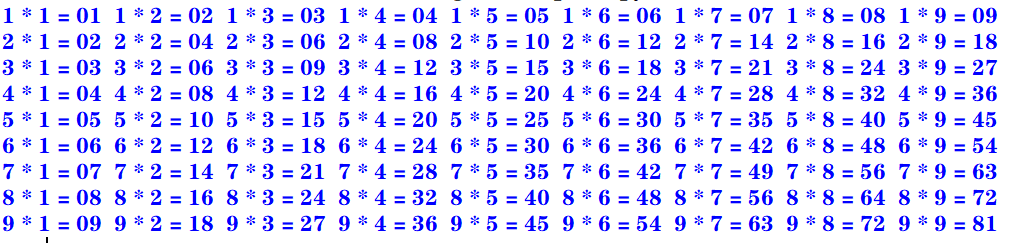下三角：
for i in range(1,10):
s = ""
for j in range(1,i+1):
s += str.format("%d * %d = %02d  " %(i, j, i*j))
print(s)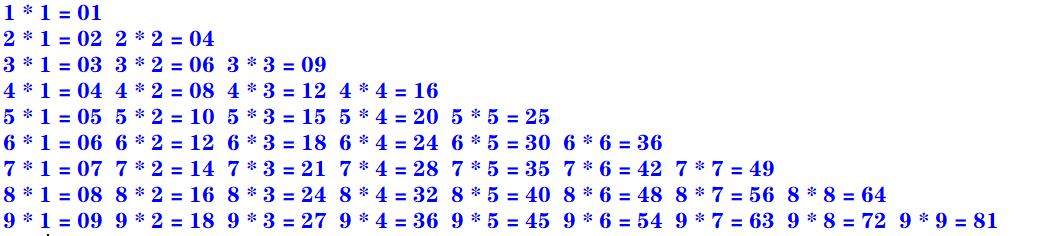上三角：
for i in range(1,10):
s = ""
for k in range(1,i):
s += "                   "
for j in range(i,10):
s += str.format("%d * %d = %02d  " %(i, j, i*j))
print(s)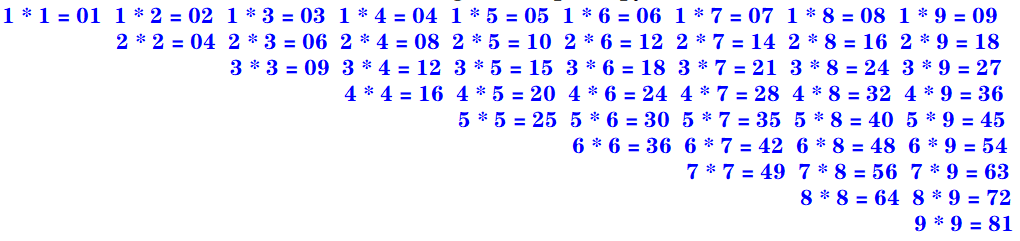三：enumerate()函数和下标元素循环示例

Python语言中的for循环直接迭代对象集合中的元素，如果需要在循环中使用索引下标访问集合元素，则可以使用内置的enumerate()函数

enumerate()函数用于将一个可遍历的数据对象（例如列表、元组或字符串）组合为一个索引序列，并返回一个可迭代对象，故在for循环当中可直接迭代下标和元素
seasons = ["Spring","Summer","Autumn","Winter"]
for i,s in enumerate(seasons,start=1):    #start默认从0开始
print("第{0}个季节：{1}".format(i,s))

运行：
第1个季节：Spring
第2个季节：Summer
第3个季节：Autumn
第4个季节：Winter

四：zip()函数和并行循环示例

如果需要并行遍历多个可迭代对象，则可以使用Python的内置函数zip()

zip()函数将多个可迭代对象中对应的元素打包成一个个元组，然后返回一个可迭代对象。如果元素的个数不一致，则返回列表的长度与最短的对象相同。
利用运算符*还可以实现将元组解压为列表
evens = [0,2,4,6,8]
odds = [1,3,5,7,9]
for e,o in zip(evens,odds):
print("{0} * {1} = {2}".format(e,o,e*o))

运行：
0 * 1 = 0
2 * 3 = 6
4 * 5 = 20
6 * 7 = 42
8 * 9 = 72

五：map()函数和循环示例

如果需要遍历可迭代对象，并使用指定函数处理对应的元素，则可以使用Python的内置函数map()

map(func,seq1[,seq2,...])

func作用于seq中的每一个元素，并将所有的调用结果作为可迭代对象返回。如果func为None，该函数的作用等同于zip()函数
计算绝对值：
>>> list(map(abs, [-1, 0, 7, -8]))
[1, 0, 7, 8]

计算乘幂：
>>> list(map(pow, range(5), range(5)))
[1, 1, 4, 27, 256]

计算ASCII码：
>>> list(map(ord, 'zgh'))
[122, 103, 104]

字符串拼接（使用了匿名函数lambda）：
>>> list(map(lambda x, y: x+y, 'zgh', '666'))
['z6', 'g6', 'h6']

选择题：1、2、3
1. 下面的Python循环体的执行次数与其他不同的是
A.
i = 0
while(i <= 10):
print(i)
i = i + 1

B.
i = 10
while(i > 0):
print(i)
i = i - 1

C.
for i in range(10):
print(i)

D.
for i in range(10,0,-1):
print(i)

答案：A
A：[0,10] 执行11次 B：[10,1] 执行10次 C：[0,9) 执行10次 D：[10,0) 执行10次
2. 执行下列Python语句将产生的结果是
x = 2; y = 2.0
if(x == y): print("Equal")
else: print("Not Equal")

A. EqualB. Not EqualC. 编译错误D. 运行时错误
答案：A

Python中的自动类型转换：
自动类型转换注意针对Number数据类型来说的当2个不同类型的数据进行运算的时候,默认向更高精度转换数据类型精度从低到高:bool int float complex关于bool类型的两个值：True 转化成整型是1；False 转化成整型是0

int类型的2转化为float类型的2.0
3. 执行下列Python语句将产生的结果是
i= 1
if(i): print(True)
else: print(False)

A. 输出1B. 输出TrueC. 输出FalseD. 编译错误
答案：B
在Python中，条件表达式最后被评价为bool值True或False。
如果表达式的结果为数值类型（0），空字符串（""），空元组（()），空列表（[]），空字典（{}），其bool值为False，否则其bool值为True
填空题：6
6. 要使语句for i in range(_,-4,-2)循环执行15次，则循环变量i的初值应当为
答案：26或者25
一开始我给的答案是26，经过评论区 亻的提醒：>>> a = 0
>>> for i in range(26, -4, -2): a+=1

>>> print(a)
15

>>> a = 0
>>> for i in range(25, -4, -2): a+=1

>>> print(a)
15

这种题目有一个规律：for i in range(x,y,z): 若循环中没有break或者continue语句， 执行次数的绝对值：result = (x-y)÷z
但实际上没有这么简单：
如果步长为 -1或者1，那么答案只有一个如果步长为 -2或者2，那么答案有两个如果步长为 -3或者3，那么答案有三个…
通过公式算出 x 之后，
如果步长为2，还要计算 (x ± 1) - z × (result-1) 的值，然后再经过琐碎的判断即可如果步长为3，还要计算 (x ± 2) - z × (result-1) 的值，……
虽然看着麻烦，但实际上是很好理解的
思考题：3~6
3. 阅读下面的Python程序，请问程序的功能是什么？
from math import sqrt

n = 0
for m in range(101,201,2):
k = int(sqrt(m))
for i in range(2, k+2):
if m % i == 0:break
if i == k + 1:
if n % 10 == 0:print()
print('%d' % m,end = " ")
n += 1

输出101到200之间的素数 每行输出10个，多余换行
运行：
101 103 107 109 113 127 131 137 139 149
151 157 163 167 173 179 181 191 193 197
199


素数（质数）是指在大于1的自然数中，除了1和它本身以外不再有其他因数的自然数。

4. 阅读下面的Python程序，请问输出的结果使什么？
n = int(input("请输入图形的行数："))
for i in range(0, n):
for j in range(0, 10 - i):print(" ", end=" ")
for k in range(0, 2 * i + 1):print(" * ", end=" ")
print("\n")

输出的是一个金字塔
运行：
请输入图形的行数：4
*

*   *   *

*   *   *   *   *

*   *   *   *   *   *   *

5. 阅读下面的Python程序，请问输出的结果使什么？程序的功能是什么？
for i in range(100,1000):
n1 = i // 100
n2 = i // 10 % 10
n3 = i % 10
if(pow(n1, 3) + pow(n2, 3) + pow(n3, 3) == i):print(i, end=" ")

输出三位数中所有的水仙花数
运行：
153 370 371 407


水仙花数 是指一个 3 位数，它的每个位上的数字的 3次幂之和等于它本身

6. 阅读下面的Python程序，请问输出的结果使什么？程序的功能是什么？
for n in range(1,1001):
total = 0; factors = []
for i in range(1, n):
if(n % i == 0):
factors.append(i)
total += i
if(total == n):print("{0} : {1}".format(n, factors))

输出1到1000的所有完数，并输出每个完数的所有因子
运行：
6 : [1, 2, 3]
28 : [1, 2, 4, 7, 14]
496 : [1, 2, 4, 8, 16, 31, 62, 124, 248]


完数 所有的真因子（即除了自身以外的约数）的和（即因子函数），恰好等于它本身

上机实践：2~14
2. 编写程序，计算1=2+3+…+100之和
使用for循环（递增）：
total = 0
for i in range(101):
total += i
print(total)

使用求和公式：
>>> (1 + 100) * 100 /2
5050.0

使用累计迭代器itertools.accumulate
>>> import itertools
>>> list(itertools.accumulate(range(1, 101)))
5050

3. 编写程序，计算10+9+8+…+1之和
使用for循环（递增）：
total = 0
for i in range(11):
total += i
print(total)

使用for循环（递减）：
total = 0
for i in range(10,0,-1):
total += i
print(total)

使用求和公式：
>>> (1 + 10) * 10 / 2
55.0

使用累计迭代器itertools.accumulate
>>> import itertools
>>> list(itertools.accumulate(range(1,11)))
55

4. 编写程序，计算1+3+5+7+…+99之和
使用for循环（递增）：
total = 0
for i in range(1,100,2):
total += i
print(total)

使用求和公式：
>>> (1 + 99) * 50 /2
2500.0

使用累计迭代器itertools.accumulate
>>> import itertools
>>> list(itertools.accumulate(range(1,100,2)))
2500

5. 编写程序，计算2+4+6+8+…+100之和
使用for循环（递增）：
total = 0
for i in range(2,101,2):
total += i
print(total)

使用求和公式：
>>> (2 + 100) * 50 / 2
2550.0

使用累计迭代器itertools.accumulate
>>> import itertools
>>> x = list(itertools.accumulate(range(2,101,2)))
>>> x[len(x)-1]
2550

6. 编写程序，使用不同的实现方法输出2000~3000的所有闰年
代码一：
for y in range(2000,3001):
if((y % 4 == 0 and y % 100 != 0) or y % 400 == 0):
print(y,end = ' ')

代码二（使用calendar模块的isleap()函数来判断）：
from calendar import isleap

for y in range(2000,3001):
if(isleap(y)):print(y,end = " ")

运行：
2000 2004 2008 2012 2016 2020 2024 2028 2032 2036 2040 2044 2048 2052 2056 2060 2064 2068 2072 2076 2080 2084 2088 2092 2096 2104 2108 2112 2116 2120 2124 2128 2132 2136 2140 2144 2148 2152 2156 2160 2164 2168 2172 2176 2180 2184 2188 2192 2196 2204 2208 2212 2216 2220 2224 2228 2232 2236 2240 2244 2248 2252 2256 2260 2264 2268 2272 2276 2280 2284 2288 2292 2296 2304 2308 2312 2316 2320 2324 2328 2332 2336 2340 2344 2348 2352 2356 2360 2364 2368 2372 2376 2380 2384 2388 2392 2396 2400 2404 2408 2412 2416 2420 2424 2428 2432 2436 2440 2444 2448 2452 2456 2460 2464 2468 2472 2476 2480 2484 2488 2492 2496 2504 2508 2512 2516 2520 2524 2528 2532 2536 2540 2544 2548 2552 2556 2560 2564 2568 2572 2576 2580 2584 2588 2592 2596 2604 2608 2612 2616 2620 2624 2628 2632 2636 2640 2644 2648 2652 2656 2660 2664 2668 2672 2676 2680 2684 2688 2692 2696 2704 2708 2712 2716 2720 2724 2728 2732 2736 2740 2744 2748 2752 2756 2760 2764 2768 2772 2776 2780 2784 2788 2792 2796 2800 2804 2808 2812 2816 2820 2824 2828 2832 2836 2840 2844 2848 2852 2856 2860 2864 2868 2872 2876 2880 2884 2888 2892 2896 2904 2908 2912 2916 2920 2924 2928 2932 2936 2940 2944 2948 2952 2956 2960 2964 2968 2972 2976 2980 2984 2988 2992 2996

7. 编写程序，计算Sn=1-3+5-7+9-11…
代码一：
n = int(input("项数："))
total = 0
flag = True
for i in range(1,2*n,2):
if(flag):
total += i
flag = False
else:
total -= i
flag = True
print(total)

代码二：
n = int(input("项数："))
total = 0
x = 2
for i in range(1,2*n,2):
total += pow(-1,x)*i
x += 1
print(total)

运行：
项数：10
-10

8. 编写程序，计算Sn=1+1/2+1/3+…
n = int(input("项数："))
total = 0.0
for i in range(1,n+1):
total += 1/i
print(total)

运行：
项数：10
2.9289682539682538

9. 编写程序，打印九九乘法表。要求输入九九乘法表的各种显示效果（上三角，下三角，矩形块等方式）
矩形块：
for i in range(1,10):
s = ""
for j in range(1,10):
s += str.format("%d * %d = %02d  " %(i, j, i*j))
print(s)下三角：
for i in range(1,10):
s = ""
for j in range(1,i+1):
s += str.format("%d * %d = %02d  " %(i, j, i*j))
print(s)上三角：
for i in range(1,10):
s = ""
for k in range(1,i):
s += "                   "
for j in range(i,10):
s += str.format("%d * %d = %02d  " %(i, j, i*j))
print(s)10. 编写程序，输入三角形的三条边，先判断是否可以构成三角形，如果可以，则进一步求三角形的周长和面积，否则报错“无法构成三角形！”
from math import sqrt

a = float(input("请输入三角形的边长a："))
b = float(input("请输入三角形的边长b："))
c = float(input("请输入三角形的边长c："))

if(a < b): a,b = b,a
if(a < c): a,c = c,a
if(b < c): b,c = c,b

if(a < 0 or b < 0 or c < 0 or b+c <= a): print("无法构成三角形！")
else:
h = (a+b+c)/2
area = sqrt(h*(h-a)*(h-b)*(h-c))
print("周长：{0}，面积：{1}".format(a+b+c,area))

运行：
请输入三角形的边长a：4
请输入三角形的边长b：3
请输入三角形的边长c：5
周长：12.0，面积：6.0

11. 编写程序，输入x，根据如下公式计算分段函数y的值。请分别用单分支语句，双分支语句结构以及条件运算语句等方法实现
y = (x2-3x)/(x+1) + 2π + sinx （x≥0 ） y = ln(-5x) + 6√(|x|+e4) - (x+1)3 (x<0)
单分支语句：
import math

x = float(input("请输入x："))
if(x >= 0):
y = (x*x - 3*x)/(x+1) + 2*math.pi + math.sin(x)
if(x < 0):
y = math.log(-5*x) + 6 * math.sqrt(abs(x) + math.exp(4)) - pow(x+1,3)

print(y)


双分支语句：
import math

x = float(input("请输入x："))
if(x >= 0):
y = (x*x - 3*x)/(x+1) + 2*math.pi + math.sin(x)
else:
y = math.log(-5*x) + 6 * math.sqrt(abs(x) + math.exp(4)) - pow(x+1,3)

print(y)

条件运算语句：
import math

x = float(input("请输入x："))
y = ((x*x - 3*x)/(x+1) + 2*math.pi + math.sin(x)) if(x >= 0) \
else (math.log(-5*x) + 6 * math.sqrt(abs(x) + math.exp(4)) - pow(x+1,3))

print(y)

运行一：
请输入x：666
668.2715406628656

运行二：
请输入x：-666
294079794.1744833

12. 编写程序，输入一元二次方程的3个系数a、b、c，求ax2+bx+c=0方程的解
import math

a = float(input("请输入系数a："))
b = float(input("请输入系数b："))
c = float(input("请输入系数c："))

delta = b*b -4*a*c

if(a == 0):
if(b == 0): print("无解")
else: print("有一个实根：",-1*c/b)
elif(delta == 0): print("有两个相等实根：x1 = x2 = ", (-1*b)/(2*a))
elif(delta > 0): print("有两个不等实根：x1 = {0}，x2 = {1}".format\
((-1*b +math.sqrt(delta))/2*a,(-1*b -math.sqrt(delta))/2*a))
elif(delta < 0): print("有两个共轭复根：x1 = {0}，x2 = {1}".format\
(complex( (-1*b)/(2*a),math.sqrt(delta*-1)/(2*a)),complex( (-1*b)/(2*a),-1*math.sqrt(delta*-1)/(2*a))))

运行一：
请输入系数a：0
请输入系数b：0
请输入系数c：10
无解

运行二：
请输入系数a：0
请输入系数b：10
请输入系数c：5
有一个实根： -0.5

运行三：
请输入系数a：1
请输入系数b：8
请输入系数c：16
有两个相等实根：x1 = x2 =  -4.0

运行四：
请输入系数a：1
请输入系数b：-5
请输入系数c：6
有两个不等实根：x1 = 3.0，x2 = 2.0

运行五：
请输入系数a：5
请输入系数b：2
请输入系数c：1
有两个共轭复根：x1 = (-0.2+0.4j)，x2 = (-0.2-0.4j)

13. 编写程序，输入整数n（n≥0）,分别利用for循环和while循环求n!
for循环
n = int(input("请输入n："))

if(n == 0): total = 1
if(n > 0):
total = 1
for i in range(n,0,-1):
total *= i

print(total)


while循环
n = int(input("请输入n："))

if(n == 0): total = 1
if(n > 0):
total = 1
while(n >= 1):
total *= n
n -= 1

print(total)

补充一个：使用累计迭代器itertools.accumulate
>>> import itertools, operator
>>> n = int(input('请输入n：'))
请输入n：7
>>> x = list(accumulate(range(1, n+1), operator.mul))
>>> x[len(x)-1]
5040

14. 编写程序，产生两个0~100（包含0和100）的随机整数a和b，求这两个整数的最大公约数和最小公倍数
现有知识点解决方法

import random

a = random.randint(0,100)
b = random.randint(0,100)
sum = a*b

print(a) #输出原来的a，b
print(b)

if(a < b): a,b = b,a

while(a%b != 0):
a,b = b,a%b

print("最大公约数：{0}，最小公倍数：{1}".format(b,sum/b))


补充：使用生成器（generate）函数：yield
>>> def func(a, b):
if(a < b): a,b = b,a
while(a%b != 0):
a,b = b,a%b
yield b

>>> import random
>>> if __name__ == '__main__':
a = random.randint(0,100)
b = random.randint(0,100)
sum = a*b
print(a,b)
t = list(iter(func(a, b)))
gcd = t[len(t)-1]
print("gcd = {0}, mcm = {1}".format(gcd, sum/gcd))

29 65
gcd = 1, mcm = 1885.0

补充：使用math模块中的gcd(x,y)函数
>>> import random
>>> import math
>>> if __name__ == '__main__':
a = random.randint(0,100)
b = random.randint(0,100)
sum = a*b
print(a,b)
gcd = math.gcd(a,b)
print("gcd = {0}, mcm = {1}".format(gcd, sum/gcd))

29 48
gcd = 1, mcm = 1392.0

案例研究：使用嵌套循环实现图像处理算法
https://blog.csdn.net/Zhangguohao666/article/details/103935185
通过图像处理算法案例，深入了解Python数据结构和基本算法流程
第四章 常用内置数据类型

几个例题
一：Python内置数据类型概述
Python中一切皆为对象，而每个对象属于某个数据类型

Python的数据类型包括：
内置的数据类型模块中定义的数据类型用户自定义的类型

四种内置的数值类型：int，float，bool，complex

int 与其他计算机语言有精度限制不同，Python中的整数位数可以为任意长度（只受限于计算机内存）。 整型对象是不可变对象。float 与其他计算机语言中的double和float对应 Python的浮点类型的精度和系统相关boolcomplex 当数值字符串中包含虚部j（或J）时即复数字面量

序列数据类型：str，tuple，bytes，list，bytearray

序列数据类型表示若干有序数据.

不可变序列数据类型：
str（字符串） 表示Unicode字符序列，例如：“zgh666” 在Python中没有独立的字符数据类型，字符即长度为1的字符串tuple（元组） 表示任意数据类型的序列，例如：(“z”,“g”,“h”,6,6,6)bytes（字节序列） 表示字节（8位）序列数据

可变序列数据类型：
list（列表） 表示可以修改的任意类型数据的序列，比如：[‘z’,‘g’,‘h’,6,6,6]bytearray（字节数组） 表示可以修改的字节（8位）数组

集合数据类型：set，frozenset

集合数据类型表示若干数据的集合，数据项目没有顺序，且不重复
set（集） 例如：{1，2，3}frozenset（不可变集）

字典数据类型：dict

字典数据类型用于表示键值对的字典 例如：{1:"zgh", 2:666}

NoneType，NotImplementedType，EllipsisType

NoneType数据类型包含唯一值None，主要用于表示空值，如没有返回值的函数的结果NotImplementedType数据类型包含唯一值NotImplemented，在进行数值运算和比较运算时，如果对象不支持，则可能返回该值EllipsisType数据类型包含唯一值Ellipsis，表示省略字符串符号...

其他数据类型

Python中一切对象都有一个数据类型，模块、类、对象、函数都属于某种数据类型 Python解释器包含内置类型， 例如： 代码对象Code objects 框架对象Frame objects 跟踪对象Traceback objects 切片对象Slice objects 静态方法对象Static method objects 类方法对象Class method objects

二：整型字面量示例

Python3.7支持使用下划线作为整数或者浮点数的千分位标记，以增强大数值的可阅读性。 二进制、八进制、十六进制则使用下划线区分4位标记

1_000_000_000  #输出1000000000

0xff_ff_ff_ff  #输出4294967295
0x_FF_FF_FF_FF  #输出4294967295

三：字符串字面量示例

两个紧邻的字符串，如果中间只有空格分隔，则自动拼接位一个字符串

'zgh' '666'  #输出'zgh666'
'zgh' + "666"   #输出'zgh666'

四：转义字符示例

转义字符后跟Unicode编码也可以表示字符
\ooo八进制Unicode码对应的字符\xhh十六进制Unicode码对应的字符

'\101'  #输出'A'
'\x41'  #输出'A'


使用r’‘或者R’'的字符串称为原始字符串，其中包含的任何字符都不进行转义

s = r'换\t行\t符\n'
s  		  #输出：'换\\t行\\t符\\n'
print(s)  #输出：换\\t行\\t符\\n

五：字符串的格式化
一：
"student number：{0}，score_average：{1}".format(2,100)
#输出：'student number：2，score_average：100'

二：
str.format("student number：{0}，score_average：{1}",2,100)
#输出：'student number：2，score_average：100'

三（兼容Python2的格式，不推荐使用）：
 "student number：%4d，score_average：%2.1f" %(2,100)
#输出：'student number：   2，score_average：100.0'

六：字符串示例，格式化输出字符串堆积的三角形

str.center()方法用于字符串两边填充str.rjust()方法用于字符串右填充

print("1".center(20))		#一行20个字符，居中对齐
print(format("121","^20"))	#一行20个字符，居中对齐
print("1".rjust(20,"*"))	#一行20个字符，右对齐，加*
print(format("121","*>20"))	#一行20个字符，右对齐，加*

运行：
         1
121
*******************1
*****************121

选择题：11
11. 关于Python字符串，下列说法错误的是
A. 字符即长度为1的字符串 B. 字符串以/0标识字符串的结束 C. 用户既可以用单引号，也可以用双引号创建字符串 D. 用三引号字符串中可以包含换行回车等特殊字符
答案：B

Python中字符串不是用\0来判断字符串结尾， 每个字符串都存有字符串的长度，通过计数来判断是否到达结尾。

虽然在c语言中\0就是来判断字符串的结尾;
填空题：4、7、8、9、10、13、21
4. Python表达式3 ** 2 ** 3的值为
答案：6561

表达式中，相同优先级的运算，从右往左

7. Python语句print(pow(-3,2),round(18.67,1),round(18.67,-1))的输出结果是
答案：9 18.7 20.0

pow()幂运算 round()四舍六入，五留双

8. Python语句print(round(123.84,0),round(123.84,-2),floor(15.5))的输出结果是
答案：124.0 100.0 15

补充：floor()是math模块中的方法，向下取整

9. Python语句print(int(‘20’,16),int(‘101’,2))的输出结果是
答案：32 5

注意：int(x,y)是指将y进制的数值x转化为10进制数

10. Python语句print(hex(16),bin(10))的输出结果是
答案：0x10 0b1010

hex(x)将十进制数x转化为十六进制，以字符串形式输出 bin(x)将十进制数x转化为二进制，以字符串形式输出

13. Python语句print(gcd(12,16),divmod(7,3))的输出结果是
答案：4 (2,1)

gcd()是math模块中的函数，求最大公约数 divmod()是内置函数，返回商和余数

21. Python语句序列 x=True;y=False;z=False;print(x or y and z) 的运行结果是
答案：True

and优先级比or高

思考题：5
5. 阅读下面的Python程序，请问输出结果是什么？
from decimal import *

ctx = getcontext()
ctx.prec = 2
print(Decimal('1.78'))#1.78
print(Decimal('1.78') + 0)#1.8
ctx.rounding = ROUND_UP
print(Decimal('1.65') + 0)#1.7
print(Decimal('1.62') + 0)#1.7
print(Decimal('-1.45') + 0)#-1.5
print(Decimal('-1.42') + 0)#-1.5
ctx.rounding = ROUND_HALF_UP
print(Decimal('1.65') + 0)#1.7
print(Decimal('1.62') + 0)#1.6
print(Decimal('-1.45') + 0)#-1.5
ctx.rounding = ROUND_HALF_DOWN
print(Decimal('1.65') + 0)#1.6
print(Decimal('-1.45') + 0)#-1.4

上机实践：2~14
2. 编写程序，格式化输出杨辉三角

杨辉三角即二项式定理的系数表，各元素满足如下条件：第一列及对角线上的元素均为1；其余每个元素等于它上一行同一列元素与前一列元素之和

我使用了一个更加精妙的规律 比如第一行为1 第二行：01 + 10 = 11 第三行：011 + 110 = 121 第四行：0121 + 1210 = 1331 。。。
def generate(numRows):
l1 = 
n = 0
while n < numRows:
print(str(l1).center(66))
l1 = [sum(t) for t in zip( + l1, l1 + )]  #利用zip函数算出每一行 如第二行 zip([0,1],[1,0])=[1,1],以此类推
n += 1
a=int(input("请输入行数"))
generate(a)

运行：
请输入行数4

[1, 1]
[1, 2, 1]
[1, 3, 3, 1]

3. 输入直角三角形的两个直角边，求三角形的周长和面积，以及两个锐角的度数。结果保留一位小数
import math

a = float(input("请输入直角三角形的直角边a："))
b = float(input("请输入直角三角形的直角边b："))
c = math.sqrt(a*a+b*b)

p = a + b + c
area = 0.5*a*b
print("三角形的周长：{0:1.1f}，面积：{1:1.1f}".format(p,area))

sina = a/c
sinb = b/c

a_degree = round(math.asin(sina) * 180 / math.pi,0)
b_degree = round(math.asin(sinb) * 180 / math.pi,0)

print("三角形直角边a的度数：{0}，b的度数：{1}".format(a_degree,b_degree))

运行：
请输入直角三角形的直角边a：3
请输入直角三角形的直角边b：4
三角形的周长：12.0，面积：6.0
三角形直角边a的度数：37.0，b的度数：53.0

4. 编程产生0~100（包含0和100）的三个随机数a、b、c，要求至少使用两种不同的方法，将三个数按从小到大的顺序排序
方法一：
import random

a = random.randint(0, 100)
b = random.randint(0, 100)
c = random.randint(0, 100)

print(str.format("原始值：{0}，{1}，{2}", a, b, c))

if(a > b): a,b = b,a
if(a > c): a,c = c,a
if(b > c): b,c = c,b

print(str.format("增序：{0}，{1}，{2}", a, b, c))

方法二（使用内置函数max、min、sum）：
import random

a = random.randint(0, 100)
b = random.randint(0, 100)
c = random.randint(0, 100)

print(str.format("原始值：{0}，{1}，{2}", a, b, c))

maxx = max(a, b, c)
minx = min(a, b, c)
median = sum([a, b, c]) - minx - maxx

print(str.format("增序：{0}，{1}，{2}", minx, median, maxx))

方法三（使用内置函数sorted）：
>>> import random
>>> a = random.randint(0,100)
>>> b = random.randint(0,100)
>>> c = random.randint(0,100)
>>> print("init value: {0} , {1} , {2}".format(a,b,c))
init value: 17 , 6 , 59
>>> sorted([a,b,c])
[6, 17, 59]

5. 编程计算有固定工资收入的党员每月所缴纳的党费。
工资基数3000元及以下者，交纳工资基数的0.5% 工资基数3000~5000元者，交纳工资基数的1% 工资基数在5000~10000元者，交纳工资基数的1.5% 工资基数超过10000元者，交纳工资基数的2%
salary = float(input("请输入有固定工资收入的党员的月工资："))
if salary <= 3000: dues = salary*0.005
elif salary <= 5000: dues = salary*0.01
elif salary <= 10000: dues = salary*0.15
else: dues = salary*0.02

print("交纳党费：",dues)

运行：
请输入有固定工资收入的党员的月工资：10001
交纳党费： 200.02

6. 编程实现袖珍计算器，要求输入两个操作数和一个操作符（+、-、*、/、%），根据操作符输出运算结果。注意/和%运算符的零异常问题
a = float(input("请输入操作数（左）："))
b = float(input("请输入操作数（右）："))
operator = input("请输入操作符（+、-、*、/、%）：")

if(b == 0 and (operator == '/' or operator == '%')):
print("分母为零，异常！")
else:
if operator == '+': result = a+b
elif operator == '-': result = a-b
elif operator == '*': result = a*b
elif operator == '/': result = a/b
elif operator == '%': result = a%b
print("{0} {1} {2}= {3}：".format(a,operator,b,result))

运行：
请输入操作数（左）：10
请输入操作数（右）：5
请输入操作符（+、-、*、/、%）：+
10.0 + 5.0= 15.0：

7. 输入三角形的3条边a、b、c，判断此3边是否可以构成三角形。若能，进一步判断三角形的性质，即为等边、等腰、直角或其他三角形
a = float(input("请输入三角形的边a："))
b = float(input("请输入三角形的边b："))
c = float(input("请输入三角形的边c："))

if(a > b): a,b = b,a
if(a > c): a,c = c,a
if(b > c): b,c = c,b

result = "三角形"
if(not(a>0 and b>0 and c>0 and a+b>c)):
result = '此三边无法构成三角形'
else:
if a == b == c: result = '等边三角形'
elif(a==b or a==c or b==c): result = '等腰三角形'
elif(a*a+b*b == c*c): result = '直角三角形'

print(result)

运行：
请输入三角形的边a：3
请输入三角形的边b：4
请输入三角形的边c：5
直角三角形

8. 编程实现鸡兔同笼问题
已知在同一个笼子里共有h只鸡和兔，鸡和兔的总脚数为f，其中h和f由用户输入，求鸡和兔各有多少只？要求使用两种方法：一是求解方程；二是利用循环进行枚举测试
h = int(input("请输入总头数："))
f = int(input("请输入总脚数："))

def fun1(h,f):
rabbits = f/2-h
chicken = h-rabbits
if(chicken < 0 or rabbits < 0): return '无解'
return chicken,rabbits

def fun2(h,f):
for i in range(0,h+1):
if(2*i + 4*(h-i) == f):return i,h-i
return '无解'

if(h>0 and f>0 and f % 2 == 0):
if fun1(h,f)=='无解':
print("无解")
else:
print("方法一：鸡：{0}，兔：{1}".format(fun1(h,f),fun1(h,f)))
print("方法二：鸡：{0}，兔：{1}".format(fun2(h,f),fun2(h,f)))
else:
print('输入的数据无意义')

运行：
请输入总头数：100
请输入总脚数：100
无解

9. 输入任意实数x，计算ex的近似值，直到最后一项的绝对值小于10-6为止
ex = 1 + x + x2/2 + x3/3! + x4/4! + … + xn/n!
x = int(input("请输入任意实数："))

e = 1
i = 1
t = 1
a = 1
while(a >= 10e-6):
t *= i
a = pow(x,i)/t
e += a
i += 1

print(e)

运行：
请输入任意实数：1
2.7182815255731922

我发现了在Python中10e-6和pow(10,-6)是有差别的，将上述代码中的10e-6改为pow(10,-6)，输出结果会有细微的差别
运行：
请输入任意实数：1
2.7182818011463845

10. 输入任意实数a（a>=0），用迭代法求x=√a，要求计算的相对偏差小于10-6
求平方根的公式：
Xn+1 = 0.5(Xn + a/Xn)
import math

a = int(input("请输入任意实数a(>=0)："))

x = a / 2
y = (x + a/x) / 2

while(abs(y-x) >= pow(10,-6)):
x = y
y = (x + a/x) / 2

print(y)

运行：
请输入任意实数a(>=0)：2
1.414213562373095

11. 即有一个数，用3除余2，用5除余3，用7除余2，请问0~1000中这样的数有哪些？
我国古代有位大将，名叫韩信。他每次集合部队，只要求部下先后按1-3，1-5，1-7报数，然后再报告一下各队每次报数的余数，他就知道到了多少人。他的这种巧妙算法被人们称作“鬼谷算”，也叫“隔墙算”，或称为“韩信点兵”，外国人还称它为“中国余数定理”。
for i in range(0,1001):
if((i % 3 == 2 )and (i % 5 == 3) and (i % 7 == 2)): print(i, end="  ")

运行：
23  128  233  338  443  548  653  758  863  968

12. 一球从100米的高度自由下落，每次落地后反弹回原高度的一半，再落下。求小球在第10次落地时共经过多少米?第10次反弹多高
规律： 第一次下落时的高度：100 第二次下落时的高度（第一次反弹的高度）：50 第三次下落时的高度（第二次反弹的高度）：25 …
n = 10

h_down = 100
h_up = 0
sum = 0
for i in range(1,n+1):
sum += h_down+h_up
h_down = h_up = h_down/2

print("小球在第十次落地时共经过：{0}米，第十次反弹高度：{1}米".format(sum,h_up))

运行：
小球在第十次落地时共经过：299.609375米，第十次反弹高度：0.09765625米

13. 猴子吃桃问题
猴子第一天摘下若干个桃子，当天吃掉一半多一个；第二天接着吃了剩下的桃子的一半多一个；以后每天都吃了前一天剩下的桃子的一半多一个。到第八天发现只剩一个桃子了。请问猴子第一天共摘了多少个桃子？
这是一个递推问题
某天所剩桃子数x 后一天所剩桃子数y = x - (x/2+1) = x/2-1
则x = 2(y+1)
result = 1
for i in range(8,0,-1):
print("第{0}天桃子数：{1}".format(i,result))
result = 2*(result+1)

运行：
第8天桃子数：1
第7天桃子数：4
第6天桃子数：10
第5天桃子数：22
第4天桃子数：46
第3天桃子数：94
第2天桃子数：190
第1天桃子数：382

14. 计算Sn = 1+11+111+…+111…111（最后一项是n个1）。n是一个随机产生的1~10（包括1和10）中的正整数
import random

n = random.randint(1,10)

x = 1
s = 0
for i in range(1,n+1):
s += x
x = 10*x+1

print("n = {0}，sn = {1}".format(n,s))

运行：
n = 6，sn = 123456

random.randint(a, b)
生成指定范围内的整数范围：[a, b]
案例研究：科学计算和数据分析
https://blog.csdn.net/Zhangguohao666/article/details/103941448
通过Python科学计算和数据分析库的安装和基本使用，了解使用Python进行科学计算的基本方法
第五章 序列数据类型

几个例题
一：Python中内置的序列数据类型

元组也称为定值表，用于存储固定不变的表列表也称为表，用于存储其值可变的表字符串是包括若干字符的序列数据，支持序列数据的基本操作字节序列数据是包括若干字节的序列。Python抓取网页时返回的页面通常为utf-8编码的字节序列。

字节序列和字符串可以直接相互转换（字节编码和解码）：
>>> s1 = b'abc'
>>> s1
b'abc'
>>> s1.decode("utf-8")
abc

>>> s2 = "中国"
>>> s2.encode("utf-8")

二：序列的切片操作示例
>>> s = 'zgh666'
>>> s
'z'
>>> s
'h'
>>> s[:3]
'zgh'
>>> s[1:3]
'gh'
>>> s[3:6]
'666'
>>> s[3:55]
'666'
>>> s[::-1]
'666hgz'
>>> s[3:2]
''
>>> s[:]
'zgh666'
>>> s[::2]
'zh6'

三：序列的连接和重复操作

通过连接操作符+可以连接两个序列，形成一个新的序列对象通过重复操作符*可以重复一个序列n次连接操作符和重复操作符也支持复合赋值运算，即：+=，*=

>>> x = 'zgh'
>>> y = '666'
>>> x + y
'zgh666'
>>> x *2
'zghzgh'
>>> x += y
>>> x
'zgh666'
>>> y *= 3
>>> y
'666666666'

四：序列的成员关系操作
innot ins.count(x) x在s中出现的次数s.index(x) x在s中第一次出现的下标
>>> s = "zgh666"
>>> 'z' in s
True
>>> 'g' not in s
False
>>> s.count('6')
3
>>> s.index('6')
3

五：序列的排序操作
sorted(iterable,key=None,reverse=False)
>>> sorted(s)
[1, 3, 5, 9]
>>> sorted(s,reverse=True)
[9, 5, 3, 1]

>>> s = 'zGhZgH'
>>> sorted(s)
['G', 'H', 'Z', 'g', 'h', 'z']
>>> sorted(s,key=str.lower)
['G', 'g', 'h', 'H', 'z', 'Z']
>>> sorted(s,key=str.lower,reverse=True)
['z', 'Z', 'h', 'H', 'G', 'g']

六：序列的拆分
变量个数与序列长度相等 若变量个数与序列的元素个数不一致，将导致ValueError
>>> data = (118,'zgh',(100,100,100))
>>> sid,name,(chinese,english,math) = data
>>> sid
118
>>> name
'zgh'
>>> chinese
100
>>> english
100
>>> math
100

变量个数与序列长度不等 如果序列长度未知，可以使用*元组变量，将多个值作为元组赋值给元组变量。在一个赋值语句中，*元组变量只允许出现一次，否则将导致SyntaxError
>>> first,second,third,*middles,last = range(10)
>>> first
0
>>> second
1
>>> third
2
>>> middles
[3, 4, 5, 6, 7, 8]
>>> last
9

>>> first,*middles,last = sorted([58,60,60,100,70,70])
>>> sum(middles)/len(middles)
65.0

使用临时变量_ 如果只需要部分数据，序列的其它位置可以使用临时变量_
>>> record = ['zgh','858990471@qq.com','17354364147','15272502101']
>>> name,_,*phone = record
>>> name
'zgh'
>>> phone
['17354364147', '15272502101']

七：使用元组字面量，tuple创建元组实例对象的实例
>>> t1 = 1,2,3
>>> t1
(1, 2, 3)

>>> t2 = (4,5,6)
>>> t2
(4, 5, 6)

>>> t3 = (9,)
>>> t3
(9,)

如果元组中只有一个项目，后面的逗号不能省略。

Python解释器把(1)解释为整数1，将(1,)解释为元组

>>> t1 = tuple()
>>> t1
()

>>> t2 = tuple("zgh666")
>>> t2
('z', 'g', 'h', '6', '6', '6')

>>> t3 = tuple(['z','g','h'])
>>> t3
('z', 'g', 'h')

八：使用列表字面量，list创建列表实例对象的实例
>>> l1 = []
>>> l1
[]

>>> l2 = ['zgh666']
>>> l2
['zgh666']

>>> l3 = [(1,2,3)]
>>> l3
[(1, 2, 3)]

>>> l1 = list()
>>> l1
[]

>>> l2 = list(b'zgh666')
>>> l2
[122, 103, 104, 54, 54, 54]

>>> l3 = list(b'aAbBcC')
>>> l3
[97, 65, 98, 66, 99, 67]

补充：列表是可变对象，故用户可以改变列表对象中元素的值，也可以通过del删除某元素
九：列表解析表达式示例
使用列表解析表达式可以简单，高效地处理一个可迭代对象，并生成结果列表
>>> [(i,i**2) for i in range(10)]
[(0, 0), (1, 1), (2, 4), (3, 9), (4, 16), (5, 25), (6, 36), (7, 49), (8, 64), (9, 81)]

>>> [i for i in range(10) if i%2==0]
[0, 2, 4, 6, 8]

>>> [(x,y,x*y) for x in range(1,4) for y in range(1,4) if x>=y]
[(1, 1, 1), (2, 1, 2), (2, 2, 4), (3, 1, 3), (3, 2, 6), (3, 3, 9)]

选择题：4、5、7、11、12
4. Python语句序列“a = (1,2,3,None,(),[]);print(len(a))”的运行结果是
>>> a = (1,2,3,None,(),[])
>>> len(a)
6

5. Python语句序列“nums = set([1,2,2,3,3,3,4]);print(len(nums))”的运行结果是
>>> nums = set([1,2,2,3,3,3,4])
>>> nums
{1, 2, 3, 4}
>>> len(nums)
4

7. Python语句序列“s1=[4,5,6];s2=s1;s1=0;print(s2)”的运行结果是
Python中变量（如s1，s2）存储在栈中，存放的是地址 [4,5,6]存储在堆中
s1 = [4,5,6]即s1存储指向堆中[4,5,6]的地址 s2 = s1地址赋值，即s2和s1都指向同一个地址 所以对列表进行修改，两者的显示都会发生变化
>>> s1 = [4,5,6]
>>> s2 = s1
>>> s1 = 0
>>> s1
[4, 0, 6]
>>> s2
[4, 0, 6]

11. Python语句序列“s={‘a’,1,‘b’,2};print(s[‘b’])”的运行结果是
A. 语法错B. ‘b’C. 1D. 2
答案：A
通过值访问集合是没有意义的，语法也不支持
>>> s ={'a',1,'b',2}
>>> print(s['b'])
Traceback (most recent call last):
File "<pyshell#29>", line 1, in <module>
print(s['b'])
TypeError: 'set' object is not subscriptable

补充：集合set是无序不重复的，是无法通过下标访问的
12. Python语句print(r"\nGood")的运行结果是
A. 新行和字符串GoodB. r"\nGood"C. \nGoodD. 字符r、新行和字符串Good
答案：C
>>> print(r"\nGood")
\nGood


r""声明原始字符串

填空题：1、5、6、12、13、14
1. Python语句序列“fruits = [‘apple’,‘banana’,‘bear’];print(fruits[-1][-1])”的运行结果是
注意：fruit[-1]是字符串’bear’ 所以：fruit[-1][-1]即'bear[-1]'
>>> fruits = ['apple','banana','pear']
>>> fruits[-1]
'pear'
>>> fruits[-1][-1]
'r'

5. Python语句 print(’%d%%%d’%(3/2,3%2)) 的运行结果是
>>> print('%d%%%d'%(3/2,3%2))
1%1

6. Python语句序列“s = [1,2,3,4];s.append([5,6]);print(len(s))”的运行结果是
答案：5
注意append()和extend()函数的区别 s.append(x)将对象x追加到s尾部 s.extend(x)将序列x追加到s尾部
append
>>> s = [1,2,3,4]
>>> s.append([5,6])
>>> s
[1, 2, 3, 4, [5, 6]]
>>> len(s)
5

extend
>>> s = [1,2,3,4]
>>> s.extend([5,6])
>>> s
[1, 2, 3, 4, 5, 6]
>>> len(s)
6

12
>>> s =('a','b','c','d','e')
>>> s
'c'
>>> s[2:3]
('c',)
>>> s[2:4]
('c', 'd')
>>> s[1::2]
('b', 'd')
>>> s[-2]
'd'
>>> s[::-1]
('e', 'd', 'c', 'b', 'a')
>>> s[-2:-1]
('d',)
>>> s[-99:-5]
()
>>> s[-99:-3]
('a', 'b')
>>> s[::]
('a', 'b', 'c', 'd', 'e')
>>> s[1:-1]
('b', 'c', 'd')

13
>>> s = [1,2,3,4,5,6]
>>> s[:1] = []
>>> s
[2, 3, 4, 5, 6]

>>> s[:2] = 'a'
>>> s
['a', 4, 5, 6]

>>> s[2:] = 'b'
>>> s
['a', 4, 'b']

>>> s[2:3] = ['x','y']
>>> s
['a', 4, 'x', 'y']

>>> del s[:1]
>>> s
[4, 'x', 'y']

14
>>> s = ['a','b']
>>> s.append([1,2])
>>> s
['a', 'b', [1, 2]]
>>> s.extend('34')
>>> s
['a', 'b', [1, 2], '3', '4']
>>> s.extend([5,6])
>>> s
['a', 'b', [1, 2], '3', '4', 5, 6]
>>> s.insert(1,7)
>>> s
['a', 7, 'b', [1, 2], '3', '4', 5, 6]
>>> s.insert(10,8)
>>> s
['a', 7, 'b', [1, 2], '3', '4', 5, 6, 8]
>>> s
['a', 7, 'b', [1, 2], '3', '4', 5, 6]
>>> s.remove('b')
>>> s
['a', 7, [1, 2], '3', '4', 5, 6]
>>> s[3:] =[]
>>> s
['a', 7, [1, 2]]
>>> s.reverse()
>>> s
[[1, 2], 7, 'a']
>>>

思考题：2、3、5
2. 阅读下面的Python语句，请问输出结果是什么？
n = int(input('请输入图形的行数：'))

for i in range(n,0,-1):
print(" ".rjust(20-i),end=' ')
for j in range(2*i-1):print(" * ",end=' ')
print('\n')

for i in range(1,n):
print(" ".rjust(19-i),end=' ')
for j in range(2*i+1):print(" * ",end=' ')
print('\n')

运行一：
请输入图形的行数：1
*

运行二：
请输入图形的行数：2
*   *   *

*

*   *   *

运行三：
请输入图形的行数：3
*   *   *   *   *

*   *   *

*

*   *   *

*   *   *   *   *

3. 阅读下面的Python语句，请问输出结果是什么？
n = int(input('请输入上（或下）三角行数：'))

for i in range(0,n):
print(" ".rjust(19-i),end=' ')
for j in range(2*i+1):print(" * ",end=' ')
print('\n')

for i in range(n-1,0,-1):
print(" ".rjust(20-i),end=' ')
for j in range(2*i-1):print(" * ",end=' ')
print('\n')

运行：
请输入上（或下）三角行数：4
*

*   *   *

*   *   *   *   *

*   *   *   *   *   *   *

*   *   *   *   *

*   *   *

*

5. 阅读下面的Python语句，请问输出结果是什么？
先看这三句：
>>> names1 = ['Amy','Bob','Charlie','Daling']
>>> names2 = names1
>>> names3 = names1[:]

毫无疑问，此时names1,names2,names3的值都是[‘Amy’,‘Bob’,‘Charlie’,‘Daling’] 但是
>>> id(names1)
2338529391368
>>> id(names2)
2338529391368
>>> id(names3)
2338529391560

names1和names2指向同一个地址 而names3指向另一个地址
然后：
>>> names2 = 'Alice'
>>> names3 = 'Ben'
>>> names1
['Alice', 'Bob', 'Charlie', 'Daling']
>>> names2
['Alice', 'Bob', 'Charlie', 'Daling']
>>> names3
['Amy', 'Ben', 'Charlie', 'Daling']

最后：
>>> sum = 0
>>> for ls in(names1,names2,names3):
if ls == 'Alice': sum+=1
if ls == 'Ben':sum+=2

>>> print(sum)
4

上机实践：2~6
2. 统计所输入字符串中单词的个数，单词之间用空格分隔
s = input("请输入字符串：")

num = 0
for i in s:
if((i >= 'a' and i <= 'z') or (i >= 'A' and i <= 'Z')):
num += 1

print("其中的单词总数：",num)

运行：
请输入字符串：zgh666 ZGH6
其中的单词总数： 6

3. 编写程序，删除一个list里面重复元素
方法一：利用set集合不重复的性质（但结果不能保证原来的顺序）
l = [1,2,2,3,3,3,4,5,6,6,6]
s = set(l)
l = list(s)
print(l)

运行：
[1, 2, 3, 4, 5, 6]

方法二：既可以去除重复项，又可以保证原来的顺序
def unique(items):
items_existed = set()
for item in items:
if item not in items_existed:
yield item

if __name__ == '__main__':
a = [1, 8, 5, 1, 9, 2, 1, 10]
a1 = unique(a)
print(list(a1))


运行结果：
[1, 8, 5, 9, 2, 10]

对代码的分析：
可以看出，unique()函数返回的并不是items_existed，而是利用了yield

在函数定义中，如果使用yield语句代替return返回一个值，则定义了一个生成器函数（generator） 生成器函数是一个迭代器，是可迭代对象，支持迭代

a1 = unique(a) 这个函数返回的实际上是一个可迭代对象 若print(a1)得到的会是：<generator object unique at 0x0000016E23AF4F48>所以，要得到去掉重复后的列表的样子，需要将可迭代对象a1放在list()中 运行：
4. 编写程序，求列表[9,7,8,3,2,1,55,6]中的元素个数、最大值、最小值，以及元素之和、平均值。请思考有几种实现方法？
内置函数：
s = [9,7,8,3,2,1,55,6]

print("元素个数：{0}，最大值：{1}，最小值：{2}，和：{3}，平均值：{4}".\
format(len(s),max(s),min(s),sum(s),sum(s)/len(s)))

直接访问元素列表（for i in s…）：
s = [9,7,8,3,2,1,55,6]

sum = 0
max = s
min = s
length = 0
for i in s:
sum += i
length += 1
if(i > max): max = i
if(i < min): min = i

print("元素个数：{0}，最大值：{1}，最小值：{2}，和：{3}，平均值：{4}".\
format(length,max,min,sum,sum/length))


间接访问列表元素（for i in range(0,len(s))…）：
s = [9,7,8,3,2,1,55,6]

sum = 0
max = s
min = s
length = len(s)
for i in range(0,length):
sum += s[i]
if(s[i] > max): max = s[i]
if(s[i] < min): min = s[i]

print("元素个数：{0}，最大值：{1}，最小值：{2}，和：{3}，平均值：{4}".\
format(length,max,min,sum,sum/length))


正序访问（i=0;while i<len(s)…）：
s = [9,7,8,3,2,1,55,6]

sum = 0
max = s
min = s
length = len(s)

i = 0
while(i < length):
sum += s[i]
if(s[i] > max): max = s[i]
if(s[i] < min): min = s[i]
i += 1

print("元素个数：{0}，最大值：{1}，最小值：{2}，和：{3}，平均值：{4}".\
format(length,max,min,sum,sum/length))


反序访问（i=len(s)-1;while i>=0…）：
s = [9,7,8,3,2,1,55,6]

sum = 0
max = s
min = s
length = len(s)

i = length-1
while(i >= 0):
sum += s[i]
if(s[i] > max): max = s[i]
if(s[i] < min): min = s[i]
i -= 1

print("元素个数：{0}，最大值：{1}，最小值：{2}，和：{3}，平均值：{4}".\
format(length,max,min,sum,sum/length))


while True：…break
s = [9,7,8,3,2,1,55,6]

sum = 0
max = s
min = s
length = len(s)

i = 0
while(True):
if(i > length-1): break
sum += s[i]
if(s[i] > max): max = s[i]
if(s[i] < min): min = s[i]
i += 1

print("元素个数：{0}，最大值：{1}，最小值：{2}，和：{3}，平均值：{4}".\
format(length,max,min,sum,sum/length))

运行：
元素个数：8，最大值：55，最小值：1，和：91，平均值：11.375

5. 编写程序，将列表[9,7,8,3,2,1,5,6]中的偶数变成它的平方，奇数保持不变
l = [9,7,8,3,2,1,5,6]

for i,value in enumerate(l):
if(value % 2 == 0):l[i] = value**2

print(l)

运行：
[9, 7, 64, 3, 4, 1, 5, 36]

6. 编写程序，输入字符串，将其每个字符的ASCII码形成列表并输出
s = input("请输入一个字符串：")
l = list()
for i in s:
l.append(ord(i))

print(l)

运行：
请输入一个字符串：zgh666
[122, 103, 104, 54, 54, 54]

案例研究：猜单词游戏
https://blog.csdn.net/Zhangguohao666/article/details/103948234
通过猜单词游戏的设计和实现，帮助读者了解使用Python系列数据类型和控制流程
第六章 输入和输出

几个例题
一：运行时提示输入密码

输入密码时，一般需要不明显，则可以使用模块getpass，以保证用户输入的密码在控制台中不回显

import getpass

else:
print('failed!')

input()#为了看到输出结果。因为执行完毕后，控制台会立马关闭


注意：上面这个代码，如果使用IDLE执行，会因为安全问题而执行失败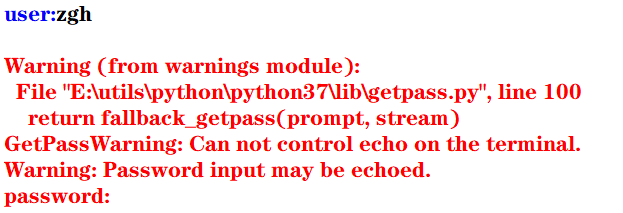但是，在控制台中执行就没问题，看输出结果（可以看到，输入的密码不会显示出来）：
user:zgh

二：重定向标准输出到一个文件的示例
这种重定向由控制台完成，而与Python本身无关。

格式： 程序 > 输出文件

其目的是将显示屏从标准输出中分离，并将输出文件与标准输出关联，即程序的执行结果将写入输出文件，而不是发送到显示屏中显示

首先准备一个test.py文件（代码如下）
import sys,random

n = int(sys.argv)
for i in range(n):
print(random.randrange(0,100))

然后在PowerShell中：python test.py 100 > scores.txt 记住，切记，一定要注意：千万能省略python，这样写./test.py 100 > scores.txt会出现问题，生成的scores文件中会没有任何内容！！！（原因未知）
然后在当前目录下，100个[0,100)范围内的的整数生成在scores.txt文件中了
三：重定向文件到标准输入

格式： 程序 < 输入文件

其目的是将控制台键盘从标准输入中分离，并将输入文件与标准输入关联，即程序从输入文件中读取输入数据，而不是从键盘中读取输入数据

准备一个average.py文件（代码如下）
import sys

total =0.0
count = 0
for line in sys.stdin:
count += 1
total += float(line)

avg = total/count
print("average:",avg)

然后问题总是不期而至， 在PowerShell中：python average.py < scores.txt，会报错，PowerShell会提示你：“<”运算符是为将来使用而保留的。 很无奈，我只能使用cmd了，然后得出结果
四：管道

格式： 程序1 | 程序2 | 程序3 | … | 程序4

其目的是将程序1的标准输出连接到程序2的标准输入， 将程序2的标准输出连接到程序3的标准输入，以此类推

例如： 打开cmd，输入python test.py 100 | average.py，其执行结果等同于上面两个例子中的命令
使用管道更加简洁，且不用创建中间文件，从而消除了输入流和输出流可以处理的数据大小的限制，执行效率更高
五：过滤器
使用操作系统实用程序more逐屏显示数据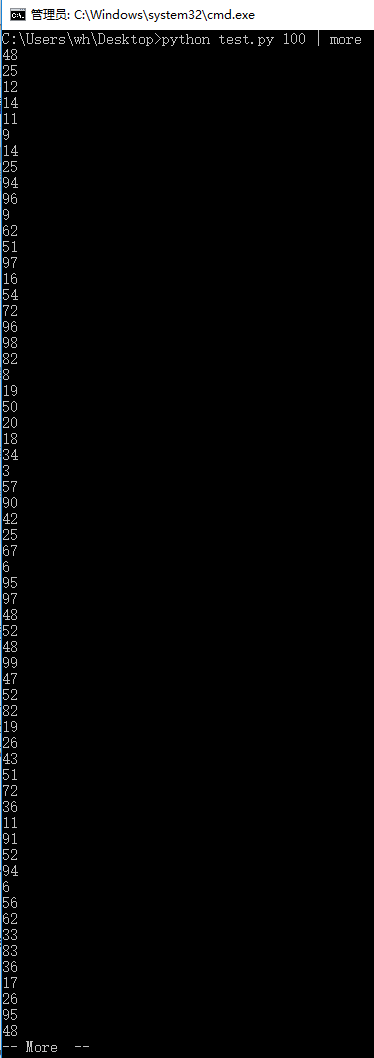使用操作系统实用程序sort排序输出数据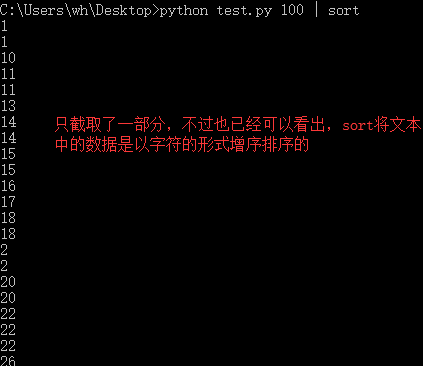more和sort都可以在一个语句中使用
填空题：1、2

print(value, ..., sep = ' ', end = '\n', file = sys.stdout, flush = False)
sep（分隔符，默认为空格）end（换行符，即输入的末尾是个啥）file（写入到指定文件流，默认为控制台sys.stdout）flush（指定是否强制写入到流）

1
>>> print(1,2,3,4,5,sep='-',end='!')
1-2-3-4-5!

2
>>> for i in range(10):
print(i,end=' ')

0 1 2 3 4 5 6 7 8 9

例题及上机实践：2~5
2. 尝试修改例6.2编写的命令行参数解析的程序，解析命令行参数所输入边长的值，计算并输出正方形的周长和面积

argparse模块用于解析命名的命令行参数，生成帮助信息的Python标准模块

例6.2：解析命令行参数所输入的长和宽的值，计算并输出长方形的面积
import argparse

parser = argparse.ArgumentParser()
parser.add_argument('--length', default = 10, type = int, help = '长度')
parser.add_argument('--width', default = 5, type = int, help = '宽度')

args = parser.parse_args()
area = args.length * args.width
print('面积 = ', area)

input()#加这一句是为了可以看到输出结果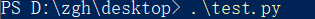输出：面积 = 50
如果在执行这个模块时，加入两个命令行参数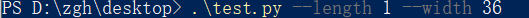输出：面积 = 36

基本上看了上面这个例子后，就可以理解argparse的用法了

本题代码：
import argparse

parser = argparse.ArgumentParser()
parser.add_argument('--length', default = 10, type = int, help = '长度')

args = parser.parse_args()
area = args.length ** 2
perimeter = 4 * args.length
print('面积 = {0}，周长 = {1}'.format(area,perimeter))

input()#加这一句是为了可以看到输出结果


在PowerShell中输入.\test.py 不给命令行参数，输出是以默认值来计算的 输出：面积 = 100，周长 = 40
给命令行参数：.\test.py --length 1 输出：面积 = 1，周长 = 4
3. 尝试修改例6.8编写读取并输出文本文件的程序，由命令行第一个参数确认所需输出的文本文件名

f = open(file, mode = 'r' , buffering = -1, encoding = None)
file是要打开或创建的文件名，如果文件不在当前路径，需指出具体路径mode是打开文件的模式，模式有： ‘r’（只读） ‘w’（写入，写入前删除就内容） ‘x’（创建新文件，如果文件存在，则导致FileExistsError） ‘a’（追加） ‘b’（二进制文件） ‘t’（文本文件，默认值） ‘+’（更新，读写）buffering表示是否使用缓存（缓存为-1，表示使用系统默认的缓冲区大小）encoding是文件的编码

例6.8：读取并输出文本文件
import sys

filename = sys.argv#就读取本文件，骚的呀皮
f = open(filename, 'r', encoding = 'utf-8')

line_no = 0
while True:
line_no += 1
if line:
print(line_no, ":", line)
else:
break
f.close()

输出（代码输出的就是本python文件）：
1 : import sys

2 :

3 : filename = sys.argv#就读取本文件，骚的呀皮

4 : f = open(filename, 'r', encoding = 'utf-8')

5 :

6 : line_no = 0

7 : while True:

8 :     line_no += 1

10 :     if line:

11 :         print(line_no, ":", line)

12 :     else:

13 :         break

14 : f.close()

15 :


本题代码：
对例题代码进行些许修改就可以了，首先将上例中的第二个语句改为：filename = sys.argv，再考虑下面怎么进行
准备一个用来测试的文件test.txt：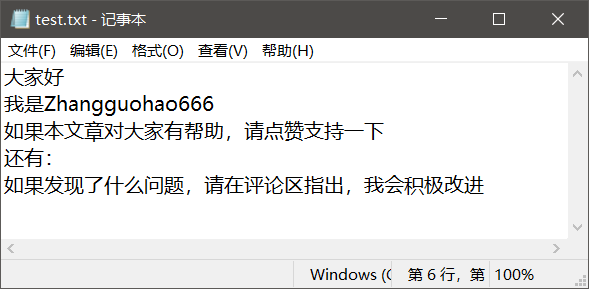对于这个文件要注意一点（你们很可能回出现这个问题！！！），win10默认创建的文本文件的字符编码是ANSI
代码怎么写，有两种：
将test.txt文本文件的编码修改为utf-8，代码如上所说 记事本方式打开test.txt文件，点击文件，点击另存为，看到下方的编码（修改为utf-8）test.txt就用默认的ANSI编码方式，再将上例代码的第三个语句修改为f = open(filename, 'r', encoding = 'ANSI')
在PowerShell中输入：./test.py test.txt 输出：
1 : ﻿大家好

2 : 我是Zhangguohao666

3 : 如果本文章对大家有帮助，请点赞支持一下

4 : 还有：

5 : 如果发现了什么问题，请在评论区指出，我会积极改进

4. 尝试修改例6.9编写利用with语句读取并输出文本文件的程序，由命令行第一个参数确认所需输出的文本文件名

为了简化操作，Python语言中与资源相关的对象可以实现上下文管理协议，可以使用with语句，确保释放资源。 with open(file,mode) as f:

例6.9：利用with语句读取并输出文本文件
import sys

filename = sys.argv

line_no = 0
with open(filename, 'r', encoding = 'utf-8') as f:
for line in f:
line_no += 1
print(line_no, ":", line)
f.close()


基本上，看这个例子，就可以上手with语句了

本题代码：
还是上一题准备的文本文件， 代码一（文本文件的编码为默认的ANSI）：
import sys

filename = sys.argv

line_no = 0
with open(filename, 'r', encoding = 'ANSI') as f:
for line in f:
line_no += 1
print(line_no, ":", line)
f.close()


代码二（将文本文件的编码修改为utf-8）：
import sys

filename = sys.argv

line_no = 0
with open(filename, 'r', encoding = 'utf-8') as f:
for line in f:
line_no += 1
print(line_no, ":", line)
f.close()


本题的输出，我再不要脸的放一次吧：
1 : ﻿大家好

2 : 我是Zhangguohao666

3 : 如果本文章对大家有帮助，请点赞支持一下

4 : 还有：

5 : 如果发现了什么问题，请在评论区指出，我会积极改进

5. 尝试修改例6.12编写标准输出流重定向的程序，从命令行第一个参数中获取n的值，然后将0-n，0-n的2倍值，2的0-n次幂的列表打印输出到out.log文件中
例6.12：从命令行第一个参数中获取n的值，然后将0-n，2的0-n次幂的列表打印输出到out.log文件中

标准输入流文件对象：sys.stdin， 默认值为sys.__stdin__标准输出流文件对象：sys.stdout， 默认值为sys.__stdout__错误输出流文件对象（标准错误流文件对象）：sys.stderr 默认值为sys.__stderr__

书中给的代码是这样的：
import sys

n = int(sys.argv)
power = 1
i = 0

f = open('out.log', 'w')
sys.stdout = f

while i <= n:
print(str(i), ' ', str(power))
power = 2*power
i += 1
sys.stdout = sys.__stdout__

如果使用的编辑器是PyCharm（现在大多数编辑器会帮你对代码进行优化和处理一些隐患），运行书中的这个代码没有问题。
但是： 若使用的编辑器是python自带的IDLE，运行这个代码有问题！
第一：out.log文件会生成，但是没有东西 （发现文件关闭不了（就是×不掉）， 确定是文件没关闭（f.close()）的原因）
第二：控制台没有输出’done’语句（估计是IDLE编辑器处理不了__stdout__这个值）
经过研究后，发现（基于IDLE编辑器）： 如果在上面的代码中加入f.close()后，该输入的东西都成功输入进out.log文件了， 但是， 还有一个问题 控制台依旧没有输出’done’语句 经过一步步的断点调试（就是手动写print） 发现sys.stdout = sys.__stdout__不会执行
然后进行改动后，就可以了，代码如下： （既然__stdout__不好使，就使用中间变量）
import sys

n = int(sys.argv)
power = 1
i = 0

output = sys.stdout
f = open('out.log', 'w')
sys.stdout = f

while i <= n:
print(str(i), ' ', str(power))
power = 2*power
i += 1

f.close()
sys.stdout = output
print('done!')#这一句是用来检测上面的代码是否成功执行



问题虽然解决，但是原因没有彻底弄清楚，求助。。。。。。

本题代码：
import sys

n = int(sys.argv)
power = 1
i = 0

output = sys.stdout
f = open('out.log', 'w')
sys.stdout = f

while i <= n:
print(str(i), ' ',  str(2*i),  ' ', str(power))
power = 2*power
i += 1

f.close()
sys.stdout = output
print('done!')#这一句是用来检测上面的代码是否成功执行


比如时输入的命令行参数是6 输出：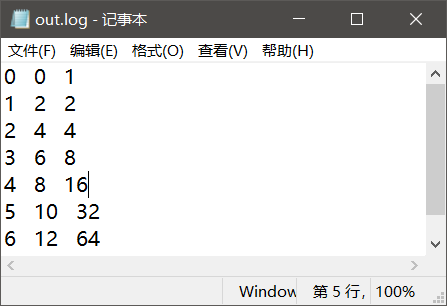案例研究：21点扑克牌游戏
https://blog.csdn.net/Zhangguohao666/article/details/103948545
通过21点扑克牌游戏的设计和实现，了解使用Python数据类型、控制流程和输入输出
第七章 错误和异常处理

Python语言采用结构化的异常处理机制捕获和处理异常

而我感觉，Python在这方面的知识点其实和Java的差不多
几个例题
一：程序的错误和异常处理
语法错误

指源代码中的拼写错误，这些错误导致Python编译器无法把Python源代码转换为字节码，故也称之为编译错误

运行时错误

在解释执行过程中产生的错误

例如：
程序中没有导入相关的模块，NameError程序中包括零除运算，ZeroDivisionError程序中试图打开不存在的文件，FileNotFoundError
逻辑错误

程序可以执行（程序运行本身不报错），但执行结果不正确。 对于逻辑错误，Python解释器无能为力，需要用户根据结果来调试判断

大部分由程序错误而产生的错误和异常一般由Python虚拟机自动抛出。另外，在程序中如果判断某种错误情况，可以创建相应的异常类的对象，并通过raise语句抛出
>>> a = -1
>>> if(a < 0): raise ValueError("数值不能为负数")

Traceback (most recent call last):
File "<pyshell#9>", line 1, in <module>
if(a < 0): raise ValueError("数值不能为负数")
ValueError: 数值不能为负数
>>>


在程序中的某个方法抛出异常后，Python虚拟机通过调用堆栈查找相应的异常捕获程序。如果找到匹配的异常捕获程序（即调用堆栈中的某函数使用try…except语句捕获处理），则执行相应的处理程序（try…except语句中匹配的except语句块）

如果堆栈中没有匹配的异常捕获程序，则Python虚拟机捕获处理异常，在控制台打印出异常的错误信息和调用堆栈，并中止程序的执行

二：try …except…else…finally
try:
可能产生异常的语句
except Exception1:
发生Exception1时执行的语句
except (Exception2,Exception3):
发生Exception2或Exception3时执行的语句
except Exception4 as e:
发生Exception4时执行的语句，Exception4的实例是e
except:
捕获其他所有异常
else:
无异常时执行的语句
finally:
不管异常发生与否都保证执行的语句

except语句可以写多个，但是要注意一点：系统是自上而下匹配发生的异常，所以用户需要将带有最具体的（即派生类程度最高的）异常类的except写在前面
三：创建自定义异常，处理应用程序中出现的负数参数的异常

自定义异常类一般继承于Exception或其子类。自定义异常类的名称一般以Error或Exception为后缀

>>> class NumberError(Exception):
def __init__(self,data):
Exception.__init__
(self,data)
self.data = data
def __str__(self):
return self.data + '：非法数值（<0）'

>>>
>>> def total(data):
total = 0
for i in data:
if i < 0: raise NumberError(str(i))
total += 1

>>>
>>> data1 = (44, 78, 90, 80, 55)
>>> print("sum: ",total(data1))
sum:  5
>>>
>>> data2 = (44, 78, 90, 80, -1)
>>> print("sum: ",total(data2))
Traceback (most recent call last):
File "<pyshell#24>", line 1, in <module>
print("sum: ",total(data2))
File "<pyshell#18>", line 4, in total
if i < 0: raise NumberError(str(i))
NumberError: -1：非法数值（<0）
>>>

四：断言处理
用户在编写程序时，在调试阶段往往需要判断代码执行过程中变量的值等信息：
用户可以使用print()函数打印输出结果也可以通过断点跟踪调试查看变量但使用断言更加灵活
assert语句和AssertionError
断言的声明：
assert <布尔表达式> 即：if __debug__: if not testexpression: raise AssertionErrorassert <布尔表达式>,<字符串表达式> 即：if __debug__: if not testexpression: raise AssertionError(data) 字符串表达式（即data）是断言失败时输出的失败消息
__debug__也是布尔值，Python解释器有两种：调试模式和优化模式
调试模式：__debug__ == True优化模式：__debug__ == False
在学习中，对于执行一个py模块（比如test.py）我们通常在cmd中这么输入python test.py，而这默认是调试模式。 如果我们要使用优化模式来禁用断言来提高程序效率，我们可以加一个运行选项-O，在控制台中这么输入python -O test.py
看一下断言的示例吧，理解一下用法：
a =int(input("a: "))
b =int(input("b: "))
assert b != 0, '除数不能为零'
c = a/b
print("a/b = ", c)

cmd出场： 输入正确数值时：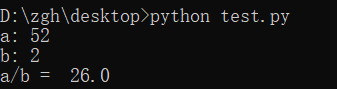输入错误数值时：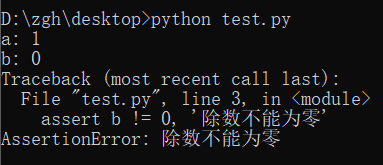禁用断言，并且输入错误数值时：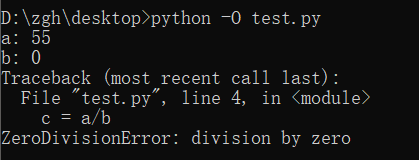案例研究：使用调试器调试Python程序
https://blog.csdn.net/Zhangguohao666/article/details/103948568
了解使用Python调试器调试程序的方法
第八章 函数和函数式编程

一些知识点总结和几个例题
Python中函数的分类：
内置函数 在程序中可以直接使用标准库函数 Python语言安装程序同时会安装若干标准库，例如math、random等第三方库函数 Python社区提供了许多其它高质量的库，在下载、安装这些库后，通过import语句可以导入库用户自定义函数
函数名为有效的标识符（命名规则为全小写字母，可以使用下划线增加可阅读性，例如my_func()）函数可以使用return返回值 如果函数体中包含return语句，则返回值， 否则不返回，即返回值为空（None），无返回值的函数相当于其它编程语言中的过程
调用函数之前程序必须先执行def语句，创建函数对象
内置函数对象会自动创建import导入模块时会执行模块中的def语句，创建模块中定义的函数Python程序结构顺序通常为import语句>函数定义>全局代码
一：产生副作用的函数，纯函数
打印等腰三角形
n = int(input("行数："))

def print_star(n):
print((" * " * n).center(50))

for i in range(1, 2*n, 2):
print_star(i)

输出：
行数：5
*
*  *  *
*  *  *  *  *
*  *  *  *  *  *  *
*  *  *  *  *  *  *  *  *

上面代码中的print_star()是一个产生副作用的函数，其副作用是向标准输出写入若干星号

副作用：例如读取键盘输入，产生输出，改变系统的状态等在一般情况下，产生副作用的函数相当于其它程序设计语言中的过程，可以省略return语句

定义计算并返回第n阶调和数（1+1/2+1/3+…+1/n）的函数，输出前n个调和数
def harmonic(n):
total = 0.0
for i in range(1, n+1):
total += 1.0/i

n = int(input("n："))

print("输出前n个调和数的值：")
for i in range(1, n+1):
print(harmonic(i))

输出：
 n：8
输出前n个调和数的值：
1.0
1.5
1.8333333333333333
2.083333333333333
2.283333333333333
2.4499999999999997
2.5928571428571425
2.7178571428571425

上面代码中的harmonic()是纯函数

纯函数：给定同样的实际参数，其返回值唯一，且不会产生其它的可观察到的副作用

注意：编写同时产生副作用和返回值的函数通常被认为是不良编程风格，但有一个例外，即读取函数。例如，input()函数既可以返回一个值，又可以产生副作用（从标准输入中读取并消耗一个字符串）
二：传递不可变对象、可变对象的引用
实际参数值默认按位置顺序依次传递给形式参数。如果参数个数不对，将会产生错误
在调用函数时：
若传递的是不可变对象（例如：int、float、bool、str对象）的引用，则如果函数体中修改对象的值，其结果实际上是创建了一个新的对象
i = 1

def func(i,n):
i += n
return i

print(i)#1
func(i,10)
print(i)#1

执行函数func()后，i依旧为1，而不是11
若传递的是可变对象（例如：list对象）的引用，则在函数体中可以直接修改对象的值
import random

def shuffle(a):
n = len(a)
for i in range(n):
r = random.randrange(i,n)
a[i],a[r] = a[r],a[i]

a = [1,2,3,4,5]
print("初始：",a)
shuffle(a)
print("调用函数后：",a)

输出：
初始： [1, 2, 3, 4, 5]
调用函数后： [1, 5, 4, 3, 2]

三：可选参数，命名参数，可变参数，强制命名参数
可选参数
在声明函数时，如果希望函数的一些参数是可选的，可以在声明函数时为这些参数指定默认值
>>> def babbles(words, times=1):
print(words * times)

>>> babbles('Hello')
Hello
>>>
>>> babbles("Hello", 2)
HelloHello
>>>

注意到一点：必须先声明没有默认值的形参，然后再声明有默认值的形参，否则报错。 这是因为在函数调用时默认是按位置传递实际参数的。
怎么理解上面那句话呢？
默认是按位置传递实际参数（如果有默认值的形参在左边，无默认值的形参在右，那么在调用函数时，你的实参该怎么传递呢？）
命名参数
位置参数：当函数调用时，实参默认按位置顺序传递形参命名参数（关键字参数）：按名称指定传入的参数 参数按名称意义明确 传递的参数与顺序无关 如果有多个可选参数，则可以选择指定某个参数值
基于期中成绩和期末成绩，按照指定的权重计算总评成绩
>>> def my_sum(mid_score, end_score, mid_rate = 0.4):
score = mid_score*mid_rate + end_score*(1-mid_rate)
print(format(score,'.2f'))

>>> my_sum(80,90)
86.00
>>> my_sum(mid_score = 80,end_score = 90)
86.00
>>> my_sum(end_score = 90,mid_score = 80)
86.00
>>>

可变参数
在声明函数时，可以通过带星号的参数（例如：def func(* param)）向函数传递可变数量的实参，调用函数时，从那一点后所有的参数被收集为一个元组在声明函数时，可以通过带双星号的参数（例如：def func(** param)）向函数传递可变数量的实参，调用函数时，从那一点后所有的参数被收集为一个字典
利用带星的参数计算各数字的累加和
>>> def my_sum(a,b,*c):
total = a+b
for i in c:
total += i

>>> print(my_sum(1,2))
3
>>> print(my_sum(1,2,3,4,5,6))
21

利用带星和带双星的参数计算各数字的累加和
>>> def my_sum(a,b,*c,**d):
total = a+b
for i in c:
total += i
for key in d:
total += d[key]

>>> print(my_sum(1,2))
3
>>> print(my_sum(1,2,3,4))
10
>>> print(my_sum(1,2,3,4,male=1,female=2))
13

强制命名参数
在带星号的参数后面声明参数会导致强制命名参数（Keyword-only），然后在调用时必须显式使用命名参数传递值因为按位置传递的参数默认收集为一个元组，传递给前面带星号的可变参数
>>> def my_sum(*, mid_score, end_score, mid_rate = 0.4):
score = mid_score*mid_rate + end_score*(1-mid_rate)
print(format(score,'.2f'))

>>> my_sum(mid_score=80,end_score=90)
86.00
>>> my_sum(end_score=90,mid_score=80)
86.00
>>> my_sum(80,90)
Traceback (most recent call last):
File "<pyshell#47>", line 1, in <module>
my_sum(80,90)
TypeError: my_sum() takes 0 positional arguments but 2 were given
>>>

四：全局语句global示例，非局部语句nonlocal示例，输出局部变量和全局变量
在函数体中可以引用全局变量，但是要为定义在函数外的全局变量赋值，需要使用global语句
pi = 2.1415926
e = 2.7182818

def my_func():
global pi
pi = 3.14
print("global pi = ", pi)
e = 2.718
print("local e = ", e)

print('module pi = ', pi)
print('module e = ', e)
my_func()
print('module pi = ', pi)
print('module e = ', e)

输出：
module pi =  2.1415926
module e =  2.7182818
global pi =  3.14
local e =  2.718
module pi =  3.14
module e =  2.7182818

在函数体中可以定义嵌套函数，在嵌套函数中如果要为定义在上级函数体的局部变量赋值，可以使用nonlocal
def outer_func():
tax_rate = 0.17
print('outer function tax rate is ',tax_rate)
def inner_func():
nonlocal tax_rate
tax_rate = 0.01
print('inner function tax rate is ',tax_rate)
inner_func()
print('outer function tax rate is ',tax_rate)

outer_func()

输出：
outer function tax rate is  0.17
inner function tax rate is  0.01
outer function tax rate is  0.01

输出局部变量和全局变量
内置函数locals()，局部变量列表内置函数globals()，全局变量列表
五：获取和设置最大递归数
在sys模块中，函数getrecursionlimit()和setrecursionlimit()用于获取和设置最大递归次数
>>> import sys
>>> sys.getrecursionlimit()
1000
>>> sys.setrecursionlimit(666)
>>> sys.getrecursionlimit()
666
>>>

六：三个有趣的内置函数：eval()、exec()、compile()
eval
对动态表达式进行求值，返回值eval(expression, globals=None, locals=None) expression是动态表达式的字符串 globals和locals是求值时使用的上下文环境的全局变量和局部变量，如果不指定，则使用当前运行上下文
>>> x = 2
>>> str_func = input("请输入表达式：")
请输入表达式：x**2+2*x+1
>>> eval(str_func)
9
>>>

exec
可以执行动态表达式，不返回值exec(str, globals=None, locals=None)
>>> exec("for i in range(10): print(i, end=' ')")
0 1 2 3 4 5 6 7 8 9
>>>

compile
编译代码为代码对象，可以提高效率compile(source, filename, mode) source为代码语句的字符串；如果是多行语句，则每一行的结尾必须有换行符\n filename为包含代码的文件 mode为编码方式，可以为'exec'（用于语句序列的执行），可以为'eval'（用于表达式求值），可以为'single'（用于单个交互语句）
>>> co = compile("for i in range(10): print(i, end=' ')", '', 'exec')
>>> exec(co)
0 1 2 3 4 5 6 7 8 9
>>>

七：map()，filter()
map(f, iterable,…)，将函数f应用于可迭代对象，返回结果为可迭代对象
示例1：
>>> def is_odd(x):
return x%2 == 1

>>> list(map(is_odd,range(5)))
[False, True, False, True, False]
>>>

示例2：
>>> list(map(abs,[1,-2,3,-4,5,-6]))
[1, 2, 3, 4, 5, 6]
>>>

示例3：
>>> list(map(str,[1,2,3,4,5]))
['1', '2', '3', '4', '5']
>>

示例4：
>>> def greater(x,y):
return x>y

>>> list(map(greater,[1,5,7,3,9],[2,8,4,6,0]))
[False, False, True, False, True]
>>>

filter(f, iterable)，将函数f应用于每个元素，然后根据返回值是True还是False决定保留还是丢弃该元素，返回结果为可迭代对象
示例1（返回个位数的奇数）：
>>> def is_odd(x):
return x%2 == 1

>>> list(filter(is_odd, range(10)))
[1, 3, 5, 7, 9]
>>>

示例2（返回三位数的回文）：
>>> list(filter(is_palindrome, range(100, 1000)))
[101, 111, 121, 131, 141, 151, 161, 171, 181, 191, 202, 212, 222, 232, 242, 252, 262, 272, 282, 292, 303, 313, 323, 333, 343, 353, 363, 373, 383, 393, 404, 414, 424, 434, 444, 454, 464, 474, 484, 494, 505, 515, 525, 535, 545, 555, 565, 575, 585, 595, 606, 616, 626, 636, 646, 656, 666, 676, 686, 696, 707, 717, 727, 737, 747, 757, 767, 777, 787, 797, 808, 818, 828, 838, 848, 858, 868, 878, 888, 898, 909, 919, 929, 939, 949, 959, 969, 979, 989, 999]
>>>

八：Lambda表达式和匿名函数

匿名函数广泛应用于需要函数对象作为参数、函数比较简单并且只使用一次的场合

格式：
lambda arg1,arg2... : <expression>

其中，arg1、arg2等为函数的参数，<expression>为函数的语句，其结果为函数的返回值
示例1（计算两数之和）：
>>> f = lambda x,y : x+y
>>> type(f)
<class 'function'>
>>> f(1,1)
2
>>>

示例2（返回奇数）：
>>> list(filter(lambda x:x%2==1, range(10)))
[1, 3, 5, 7, 9]
>>>

示例3（返回非空元素）：
>>> list(filter(lambda s:s and s.strip(), ['A', '', 'B', None, 'C', ' ']))
['A', 'B', 'C']
>>>

补充：
strip()用来去除头尾字符、空白符（\n,\r,\t,’’,即换行、回车、制表、空格）lstrip()用来去除开头字符、空白符rstrip()用来去除结尾字符、空白符
再补充一点：
\n到下一行的开头\r回到这一行的开头
示例4（返回大于0的元素）：
>>> list(filter(lambda x:x>0, [1,0,-2,8,5]))
[1, 8, 5]
>>>

示例5（返回元素的平方）：
>>> list(map(lambda x:x*x, range(10)))
[0, 1, 4, 9, 16, 25, 36, 49, 64, 81]
>>>

九：operator模块和操作符函数

Python内置操作符的函数接口，它定义了对应算术和比较等操作的函数，用于map()、filter()等需要传递函数对象作为参数的场合，可以直接使用而不需要使用函数定义或者Lambda表达式，使得代码更加简洁

示例1（concat(x,y)对应于x+y）：
>>> import operator
>>> a = 'hello'
>>>> operator.concat(a, ' world')
'hello world'

实例2（operator.gt对应于操作符>）：
>>> import operator
>>> list(map(operator.gt, [1,5,7,3,9],[2,8,4,6,0]))
[False, False, True, False, True]
>>>

十：functools.reduce()，偏函数functools.partial()，sorted()
functools.reduce()

functools.reduce(func, iterable[, iterable[, initializer]])
使用指定的带两个参数的函数func对一个数据集合的所有数据进行下列操作：使用第一个和第二个数据作为参数用func()函数运算，得到的结果再与第三个数据作为参数用func()函数运算，依此类推，最后得到一个结果可选的initialzer为初始值

示例：
>>> import functools,operator
15
25
5050
>>>
>>> functools.reduce(operator.mul, range(1,11))
3628800

偏函数functools.partial()

functools.partial(func, *arg, **keywords)
通过把一个函数的部分参数设置为默认值的方式返回一个新的可调用（callable）的partial对象主要用于设置预先已知的参数，从而减少调用时传递参数的个数

示例（2的n次方）：
>>> import functools,math
>>> pow2 = functools.partial(math.pow, 2)
>>> list(map(pow2, range(11)))
[1.0, 2.0, 4.0, 8.0, 16.0, 32.0, 64.0, 128.0, 256.0, 512.0, 1024.0]
>>>

十一：sorted()

sorted(iterable, *, key=None, reverse=False)
iterable是待排序的可迭代对象key是比较函数（默认为None，按自然顺序排序）reverse用于指定是否逆序排序

示例1（数值。默认自然排序）：
>>> sorted([1,6,4,-2,9])
[-2, 1, 4, 6, 9]
>>> sorted([1,6,4,-2,9], reverse=True)
[9, 6, 4, 1, -2]
>>> sorted([1,6,4,-2,9], key=abs)
[1, -2, 4, 6, 9]

示例2（字符串，默认按字符串字典序排序）：
>>> sorted(['Dod', 'cat', 'Rabbit'])
['Dod', 'Rabbit', 'cat']
>>> sorted(['Dod', 'cat', 'Rabbit'], key=str.lower)
['cat', 'Dod', 'Rabbit']
>>> sorted(['Dod', 'cat', 'Rabbit'], key=len)
['Dod', 'cat', 'Rabbit']

示例3（元组，默认按元组的第一个元素排序）：
>>> sorted([('Bob', 75), ('Adam', 92), ('Lisa', 88)])
[('Adam', 92), ('Bob', 75), ('Lisa', 88)]
>>> sorted([('Bob', 75), ('Adam', 92), ('Lisa', 88)], key=lambda t:t)
[('Bob', 75), ('Lisa', 88), ('Adam', 92)]

十二：函数装饰器
这玩意就很有意思了，很Java语言中的注解是很相像的
示例1：
import time,functools

def timeit(func):
def wrapper(*s):
start = time.perf_counter()
func(*s)
end = time.perf_counter()
print('运行时间：', end - start)
return wrapper

@timeit
def my_sum(n):
sum = 0
for i in range(n): sum += i
print(sum)

if __name__ == '__main__':
my_sum(10_0000)

结果：
4999950000
运行时间： 0.013929100000000028

怎么理解上面的代码呢？
首先，timeit()返回的是wrapper，而不是执行（没有小括号）@timeit相当于，在调用my_sum()的前一刻，会执行这么个语句：my_sum = timeit(my_sum)
示例2：
def makebold(fn):
def wrapper(*s):
return "<b>" + fn(*s) + "</b>"
return wrapper

def makeitalic(fn):
def wrapper(*s):
return "<i>" + fn(*s) + "</i>"
return wrapper

@makebold
@makeitalic
def htmltags(str1):
return str1

print(htmltags('Hello'))


输出：
<b><i>Hello</i></b>

选择题：1~5
1
>>> print(type(lambda:None))
<class 'function'>

2
>>> f = lambda x,y:x*y
>>> f(12, 34)
408

3
>>> f1 = lambda x:x*2
>>> f2 = lambda x:x**2
>>> print(f1(f2(2)))
8

4
>>> def f1(p, **p2):
print(type(p2))

>>> f1(1, a=2)
<class 'dict'>

5
>>> def f1(a,b,c):
print(a+b)

>>> nums = (1,2,3)
>>> f1(*nums)
3

思考题：4~11
4
>>> d = lambda p:p*2
>>> t = lambda p:p*3
>>> x = 2
>>> x = d(x)
>>> x = t(x)
>>> x = d(x)
>>> print(x)
24

5
>>> i = map(lambda x:x**2, (1,2,3))
>>> for t in i:
print(t, end=' ')

1 4 9

6
>>> def f1():
"simple function"
pass

>>> print(f1.__doc__)
simple function

7
>>> counter = 1
>>> num = 0
>>> def TestVariable():
global counter
for i in (1, 2, 3) : counter += 1
num = 10

>>> TestVariable()
>>> print(counter, num)
4 0

8
>>> def f(a,b):
if b==0 : print(a)
else : f(b, a%b)

>>> print(f(9,6))
3
None

求最大公约数
9
>>> def aFunction():
"The quick brown fox"
return 1

>>> print(aFunction.__doc__[4:9])
quick

10
>>> def judge(param1, *param2):
print(type(param2))
print(param2)

>>> judge(1, 2, 3, 4, 5)
<class 'tuple'>
(2, 3, 4, 5)

11
>>> def judge(param1, **param2):
print(type(param2))
print(param2)

>>> judge(1, a=2, b=3, c=4, d=5)
<class 'dict'>
{'a': 2, 'b': 3, 'c': 4, 'd': 5}

上机实践：2~5
2. 编写程序，定义一个求阶乘的函数fact(n)，并编写测试代码，要求输入整数n（n>=0）。请分别使用递归和非递归方式实现
递归方式：
def fact(n):
if n == 0 :
return 1
return n*fact(n-1)

n = int(input("请输入整数n（n>=0）："))
print(str(n)+" ! =  " + str(fact(n)))


非递归方式：
def fact(n):
t = 1
for i in range(1,n+1):
t *= i
return t

n = int(input("请输入整数n（n>=0）："))
print(str(n)+" ! =  " + str(fact(n)))


输出：
请输入整数n（n>=0）：5
5 ! =  120

3. 编写程序，定义一个求Fibonacci数列的函数fib(n)，并编写测试代码，输出前20项（每项宽度5个字符位置，右对齐），每行输出10个。请分别使用递归和非递归方式实现
递归方式：
def fib(n):
if (n == 1 or n == 2):
return 1
return fib(n-1)+fib(n-2)

for i in range(1,21):
print(str(fib(i)).rjust(5,' '),end = ' ')
if i %10 == 0:
print()

非递归方式：
def fib(n):
if (n == 1 or n == 2):
return 1
n1 = n2 = 1
for i  in range(3,n+1):
n3 = n1+n2
n1 = n2
n2 = n3
return n3

for i in range(1,21):
print(str(fib(i)).rjust(5,' '),end = ' ')
if i %10 == 0:
print()

输出：
    1     1     2     3     5     8    13    21    34    55
89   144   233   377   610   987  1597  2584  4181  6765

4. 编写程序，利用可变参数定义一个求任意个数数值的最小值的函数min_n(a,b,*c)，并编写测试代码。例如对于“print(min_n(8, 2))”以及“print(min_n(16, 1, 7, 4, 15))”的测试代码
def min_n(a,b,*c):
min_number = a if(a < b) else b
for n in c:
if n < min_number:
min_number = n
return min_number

print(min_n(8, 2))
print(min_n(16, 1, 7, 4, 15))

输出：
2
1

5. 编写程序，利用元组作为函数的返回值，求序列类型中的最大值、最小值和元素个数，并编写测试代码，假设测试代码数据分别为s1=[9, 7, 8, 3, 2, 1, 55, 6]、s2=[“apple”, “pear”, “melon”, “kiwi”]和s3="TheQuickBrownFox"
def func(n):
return (max(n),min(n),len(n))

s1=[9, 7, 8, 3, 2, 1, 55, 6]
s2=["apple", "pear", "melon", "kiwi"]
s3="TheQuickBrownFox"

for i in (s1,s2,s3):
print("list = ", i)
t = func(i)
print("最大值 = {0}，最小值 = {1}，元素个数 = {2}".format(t, t, t))

输出：
list =  [9, 7, 8, 3, 2, 1, 55, 6]
最大值 = 55，最小值 = 1，元素个数 = 8
list =  ['apple', 'pear', 'melon', 'kiwi']
最大值 = pear，最小值 = apple，元素个数 = 4
list =  TheQuickBrownFox
最大值 = x，最小值 = B，元素个数 = 16

案例研究：井字棋游戏
https://blog.csdn.net/Zhangguohao666/article/details/103280740
了解Python函数的定义和使用

由于本文的内容太多了，导致了两个很不好的结果， 一是：在网页中打开本篇博客的加载时间太长了，明显的卡顿很影响阅读体验； 二是：本人在对本篇文章进行更新或者修改内容时，卡的要死。 遂， 将本文第八章后面的很多内容拆分到新的文章中，望大家理解

第九章 面向对象的程序设计

《Python程序设计与算法基础教程（第二版）》江红 余青松，第九章课后习题答案
第十章 模块和客户端

《Python程序设计与算法基础教程（第二版）》江红 余青松，第十章课后习题答案
第十一章 算法与数据结构基础

《Python程序设计与算法基础教程（第二版）》江红 余青松，第十一章课后习题答案
第十二章 图形用户界面

我对图形用户界面基本无兴趣，无特殊情况，基本不打算碰这方面内容
案例研究：简易图形用户界面计算器
Python实现简易图形用户界面计算器
第十三章 图形绘制

与上一章相同，我对于图形绘制的兴趣也基本没有，尝试做了2-7题，就完全没兴趣做下去了
图形绘制模块：tkinter
2. 参考例13.2利用Canvas组件创建绘制矩形的程序，尝试改变矩形边框颜色以及填充颜色
from tkinter import *

root = Tk()
c = Canvas(root, bg = 'white', width = 130, height = 70)
c.pack()

c.create_rectangle(10, 10, 60, 60, fill = 'red')
c.create_rectangle(70, 10, 120, 60, fill = 'green', outline = 'blue', width = 5)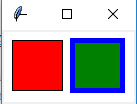创建画布对象：
root = Tk() 创建一个Tk根窗口组件rootc = Canvas(root, bg = 'white', width = 130, height = 70) 创建大小为200 * 100、背景颜色为白色的画布c.pack() 调用组件pack()方法，调整其显示位置和大小
绘制矩形：
c.create_rectangle(x0, y0, x1, y1, option, ...)

（x0，y0）是左上角的坐标（x1，y1）是右下角的坐标c.create_rectangle(70, 10, 120, 60, fill = 'green', outline = 'blue', width = 5) 用蓝色边框、绿色填充矩形，边框宽度为5
3. 参考例13.3利用Canvas组件创建绘制椭圆的程序，尝试修改椭圆边框样式、边框颜色以及填充颜色
from tkinter import *

root = Tk()
c = Canvas(root, bg = 'white', width = 280, height = 70)
c.pack()

c.create_oval(10, 10, 60, 60, fill = 'green')
c.create_oval(70, 10, 120, 60, fill = 'green', outline = 'red', width = 5)
c.create_oval(130, 25, 180, 45, dash = (10,))
c.create_oval(190, 10, 270, 50, dash = (1,), width = 2)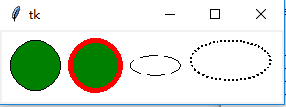绘制椭圆
c.create_oval(x0, y0, x1, y1, option, ...)

（x0，y0）是左上角的坐标（x1，y1）是右下角的坐标c.create_oval(70, 10, 120, 60, fill = 'green', outline = 'red', width = 5) 绿色填充、红色边框，宽度为5c.create_oval(130, 25, 180, 45, dash = (10,)) 虚线椭圆
4. 参考例13.4利用Canvas组件创建绘制圆弧的程序，尝试修改圆弧样式、边框颜色以及填充颜色
from tkinter import *

root = Tk()
c = Canvas(root, bg = 'white', width = 250, height = 70)
c.pack()

c.create_arc(10, 10, 60, 60, style = ARC)
c.create_arc(70, 10, 120, 60, style = CHORD)
c.create_arc(130, 10, 180, 60, style = PIESLICE)
for i in range(0, 360, 60):
c.create_arc(190, 10, 240, 60, fill = 'green', outline = 'red', start = i, extent = 30)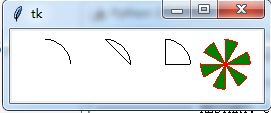绘制圆弧：
c.create_arc(x0, y0, x1, y1, option, ...)

（x0，y0）是左上角的坐标（x1，y1）是右下角的坐标选项start（开始角度，默认为0）和extend（圆弧角度，从start开始逆时针旋转，默认为90度）决定圆弧的角度范围选项start用于设置圆弧的样式
5. 参考例13.5利用Canvas组件创建绘制线条的程序，尝试修改线条样式和颜色
from tkinter import *

root = Tk()
c = Canvas(root, bg = 'white', width = 250, height = 70)
c.pack()

c.create_line(10, 10, 60, 60, arrow = BOTH, arrowshape = (3, 4, 5))
c.create_line(70, 10, 95, 10, 120, 60, fill = 'red')
c.create_line(130, 10, 180, 10, 130, 60, 180, 60, fill = 'green', width = 10, arrow = BOTH, joinstyle = MITER)
c.create_line(190, 10, 240, 10, 190, 60, 240, 60, width = 10)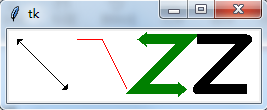绘制线条：
c.create_line(x0, y0, x1, y1, ..., xn, yn, option, ...)

（x0，y0）,（x1，y1），…，（xn，yn）是线条上各个点的坐标
6. 参考例13.6利用Canvas组件创建绘制多边形的程序，尝试修改多边形的形状、线条样式和填充颜色
from tkinter import *

root = Tk()
c = Canvas(root, bg = 'white', width = 250, height = 70)
c.pack()

c.create_polygon(35, 10, 10, 60, 60, 60, fill = 'red', outline = 'green')
c.create_polygon(70, 10, 120, 10, 120, 60, fill = 'white', outline = 'blue')
c.create_polygon(130, 10, 180, 10, 180, 60, 130, 60, outline = 'blue')
c.create_polygon(190, 10, 240, 10, 190, 60, 240, 60, fill = 'white', outline = 'black')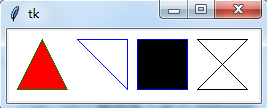绘制多边形：
c.create_polygon(x0, y0, x1, y1, ..., option, ...)

（x0，y0）,（x1，y1），…，（xn，yn）是多边形上各个顶点的坐标
7. 参考例13.7利用Canvas组件创建绘制字符串和图形的程序，绘制y = cos(x) 的图形
绘制字符串：
c.create_text(x, y, option, ...)

（x，y）是字符串放置的中心位置
y = sin(x)
from tkinter import *
import math

WIDTH, HEIGHT = 510, 210
ORIGIN_X, ORIGIN_Y = 2, HEIGHT/2 #原点

SCALE_X, SCALE_Y = 40, 100 #x轴、y轴缩放倍数
ox, oy = 0, 0
x, y = 0, 0
arc = 0 #弧度
END_ARC = 360 * 2 #函数图形画两个周期

root = Tk()
c = Canvas(root, bg = 'white', width = WIDTH, height = HEIGHT)
c.pack()

c.create_text(200, 20, text = 'y = sin(x)')
c.create_line(0, ORIGIN_Y, WIDTH, ORIGIN_Y)
c.create_line(ORIGIN_X, 0, ORIGIN_X, HEIGHT) #绘制x轴，y轴
for i in range(0, END_ARC+1, 10):
arc = math.pi * i / 180
x = ORIGIN_X + arc * SCALE_X
y = ORIGIN_Y - math.sin(arc) * SCALE_Y
c.create_line(ox, oy, x, y)
ox, oy = x, y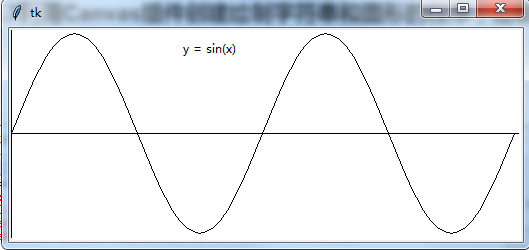y = cos(x)
from tkinter import *
import math

WIDTH, HEIGHT = 510, 210
ORIGIN_X, ORIGIN_Y = 2, HEIGHT/2 #原点

SCALE_X, SCALE_Y = 40, 100 #x轴、y轴缩放倍数
ox, oy = 0, 0
x, y = 0, 0
arc = 0 #弧度
END_ARC = 360 * 2 #函数图形画两个周期

root = Tk()
c = Canvas(root, bg = 'white', width = WIDTH, height = HEIGHT)
c.pack()

c.create_text(200, 20, text = 'y = cos(x)')
c.create_line(0, ORIGIN_Y, WIDTH, ORIGIN_Y)
c.create_line(ORIGIN_X, 0, ORIGIN_X, HEIGHT)
for i in range(0, END_ARC+1, 10):
arc = math.pi * i / 180
x = ORIGIN_X + arc * SCALE_X
y = ORIGIN_Y - math.cos(arc) * SCALE_Y
c.create_line(ox, oy, x, y)
ox, oy = x, y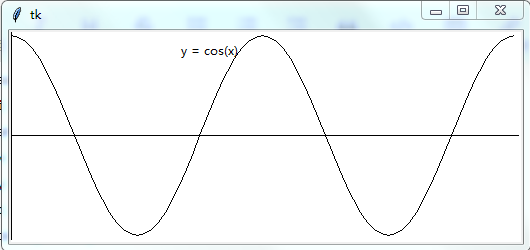图形绘制模块：turtle
…

后面章节内容：未完待续…
第十四章 数值日期和时间处理

第十五章 字符串和文本处理

第十六章 文件和数据交换

第十七章 数据访问

第十八章 网络编程和通信

第十九章 并行计算：进程、线程和协程

第二十章 系统管理
展开全文• Python语言中的缩进在程序中长度统一且强制使用，只要统一即可，一定是4个空格（尽管这是惯例） IPO模型指：Input Process Output 字符串的正向递增和反向递减序号体系：正向是从左到右，0到n-1，反向是从右到左...
测验1：Python基本语法元素
知识点概要：
普遍认为Python语言诞生于1991年Python语言中的缩进在程序中长度统一且强制使用，只要统一即可，不一定是4个空格（尽管这是惯例）IPO模型指：Input Process Output字符串的正向递增和反向递减序号体系：正向是从左到右，0到n-1，反向是从右到左，-1到-n，举例
str = "csdn"
#str就表示字符串c, str[-1]表示"n"

Python的合法命名规则：命名包含字母，数字，下划线，但是首字符不能是数字Python中获得用户输入的方式为：input()Python中的保留字：type不是，是内置函数，def elif import 都是保留字Python的数据类型有整数、列表、字符串等，但是不包括实数，实数是数学概念，在Python中对应着浮点数保留字if-elif-else用于表示分支结构，in用来进行成员判断print()格式化输出，控制浮点数的小数点后两位输出应为：print("{:.2f}".format(XX)) :.2f哪一个都不能少
编程测试：
Hello World 的条件输出：获得用户输入的一个整数，参考该整数值，打印输出"Hello World"，要求：‪‬‪‬‪‬‪‬‪‬‮‬‪‬‫‬‪‬‪‬‪‬‪‬‪‬‮‬‭‬‫‬‪‬‪‬‪‬‪‬‪‬‮‬‫‬‫‬‪‬‪‬‪‬‪‬‪‬‮‬‫‬‮‬‪‬‪‬‪‬‪‬‪‬‮‬‫‬‫‬‪‬‪‬‪‬‪‬‪‬‮‬‫‬‮‬ 如果输入值是0，直接输出"Hello World"‪‬‪‬‪‬‪‬‪‬‮‬‪‬‫‬‪‬‪‬‪‬‪‬‪‬‮‬‭‬‫‬‪‬‪‬‪‬‪‬‪‬‮‬‫‬‫‬‪‬‪‬‪‬‪‬‪‬‮‬‫‬‮‬‪‬‪‬‪‬‪‬‪‬‮‬‫‬‫‬‪‬‪‬‪‬‪‬‪‬‮‬‫‬‮‬ 如果输入值大于0，以两个字符一行方式输出"Hello World"（空格也是字符）‪‬‪‬‪‬‪‬‪‬‮‬‪‬‫‬‪‬‪‬‪‬‪‬‪‬‮‬‭‬‫‬‪‬‪‬‪‬‪‬‪‬‮‬‫‬‫‬‪‬‪‬‪‬‪‬‪‬‮‬‫‬‮‬‪‬‪‬‪‬‪‬‪‬‮‬‫‬‫‬‪‬‪‬‪‬‪‬‪‬‮‬‫‬‮‬ 如果输入值小于0，以垂直方式输出"Hello World"
# eval()函数可以将默认输入的字符串去掉双引号并进行表达式运算，如输入500+20，默认
#得到的输入为一个字符串“500+20”，但是使用eval()函数我们得到的是一个整型数字：520
Number = eval(input())
if Number == 0:
print("Hello World")
elif Number > 0:
print("He\nll\no \nWo\nrl\nd")
else:
for c in "Hello World":
print(c)

数值运算：获得用户输入的一个字符串，格式如下：M OP N ，其中，M和N是任何数字，OP代表一种操作，表示为如下四种：+, -, *, /（加减乘除）‪‬‪‬‪‬‪‬‪‬‮‬‪‬‫‬‪‬‪‬‪‬‪‬‪‬‮‬‭‬‫‬‪‬‪‬‪‬‪‬‪‬‮‬‫‬‫‬‪‬‪‬‪‬‪‬‪‬‮‬‫‬‮‬‪‬‪‬‪‬‪‬‪‬‮‬‫‬‫‬‪‬‪‬‪‬‪‬‪‬‮‬‫‬‮‬根据OP，输出M OP N的运算结果，统一保存小数点后2位。‪‬‪‬‪‬‪‬‪‬‮‬‪‬‫‬‪‬‪‬‪‬‪‬‪‬‮‬‭‬‫‬‪‬‪‬‪‬‪‬‪‬‮‬‫‬‫‬‪‬‪‬‪‬‪‬‪‬‮‬‫‬‮‬‪‬‪‬‪‬‪‬‪‬‮‬‫‬‫‬‪‬‪‬‪‬‪‬‪‬‮‬‫‬‮‬ 注意：M和OP、OP和N之间可以存在多个空格，不考虑输入错误情况。
print("{:.2f}".format(eval(input())))

测验2：Python基本图形绘制
知识点概要：
正确引用turtle库的方式：
import turtle
#t是别名,可以更换其他名称
import turtle as t
from turtle import setup
from turtle import *

import setup from turtle是不正确的
turtle库是一个直观有趣的图形绘制函数库，最早成功应用于LOGO编程语言，turtle绘图体系以水平右侧为绝对方位的0度，turtle坐标系的原点****默认在屏幕正中间turtle.circle(-90,90)表示绘制一个半径为90像素的弧形，圆心在小海龟当前行进的右侧
# circle(x,y)表示以x长度为半径，y为角度，
#当前方向左侧x处为圆心画圆，其中x,y都可以是负数，相应取反
#当前方向是水平向右的，对应直角坐标系中的x轴正方向
#x为正，则圆心在y轴正方向上，y为正，逆时针画圆，圆弧角度为y
#x为负则相反，圆心在y轴负方向上，y为正，顺时针画圆，圆弧角度为y

turtle.seth(to_angle)函数的作用是设置小海龟当前行进方向为to_angle，to_angle是角度的整数值turtle.fd(distance)函数的作用是向小海龟当前行进方向前进distance距离turtle.pensize(size)函数的作用是改变画笔的宽度为size像素turtle**.circle**()函数不能绘制椭圆形turtle.circle(x,y)函数绘制半圆，第二个参数y是180的奇数倍turtle.penup()的别名有turtle.pu(),turtle.up()turtle.colormode()的作用是设置画笔RGB颜色的表示模式turtle.width()和turtle.pensize()都可以用来设置画笔尺寸turtle.pendown()只是放下画笔，并不绘制任何内容改变turtle画笔的运行方向有left()、right()和seth()函数，bk()只能后退，但是不改变方向turtle.done()用来停止画笔绘制，但绘图窗体不关闭，建议在每个turtle绘图最后增加turtle.done()循环相关保留字是：for…in和while，def用于定义函数
编程测试：
turtle八边形绘制：使用turtle库，绘制一个八边形
import turtle as t
t.pensize(2)
for i in range(8):
t.fd(100)
t.left(45)

turtle八角图形绘制：使用turtle库，绘制一个八角图形
import turtle as t
t.pensize(2)
for i in range(8):
t.fd(150)
t.left(135)

测验3：基本数据类型
知识点概要：
pow(x,0.5)能够计算x的平方根，计算负数的平方根将产生复数字符串.strip()方法的功能是去掉字符串两侧指定的字符字符串.split()方法的功能是按照指定字符分隔字符串为数组字符串.repalce()方法的功能是替换字符串中特定字符+操作符用来连接两个字符串序列字符串是一个连续的字符序列，使用\n可以实现打印字符信息的换行val = pow(2,1000) #返回val结果的长度值要使用 len(str(val)),因为整型没有len()方法，要通过str()函数 #将数字类型转换为字符串正确引用time库的方式如下：
import time
from time import strftime
from time import *

Python语言的整数类型表示：十进制（一般表示）二进制（0b或0B开头）八进制（0o或0O开头）十六进制（0x或0X开头）%运算符的意思是取余数字符串切片操作：s[N:M]，从N到M，但是不包括M
name="Python语言程序设计课程"
print(name,name[2:-2],name[-1])
#输出结果为：P thon语言程序设计 程

print("{0:3}".format('PYTHON'))代码执行的结果是PYTHON，{0:3}表示输出的宽度是3，但是如果字符串长度超过3就以字符串长度显示
编程测试：
平方根格式化：获得用户输入的一个整数a，计算a的平方根，保留小数点后3位，并打印输出。‪‬‪‬‪‬‪‬‪‬‮‬‪‬‫‬‪‬‪‬‪‬‪‬‪‬‮‬‭‬‫‬‪‬‪‬‪‬‪‬‪‬‮‬‫‬‫‬‪‬‪‬‪‬‪‬‪‬‮‬‫‬‮‬‪‬‪‬‪‬‪‬‪‬‮‬‫‬‫‬‪‬‪‬‪‬‪‬‪‬‮‬‫‬‮‬输出结果采用宽度30个字符、右对齐输出、多余字符采用加号(+)填充，‪‬‪‬‪‬‪‬‪‬‮‬‪‬‫‬‪‬‪‬‪‬‪‬‪‬‮‬‭‬‫‬‪‬‪‬‪‬‪‬‪‬‮‬‫‬‫‬‪‬‪‬‪‬‪‬‪‬‮‬‫‬‮‬‪‬‪‬‪‬‪‬‪‬‮‬‫‬‫‬‪‬‪‬‪‬‪‬‪‬‮‬‫‬‮‬如果结果超过30个字符，则以结果宽度为准
a = eval(input())
print("{:+>30.3f}".format(a**0.5))
# +是填充字符 >是右对齐 30是宽度 .3f是保留小数点后3位
#若平凡根后是一个复数，复数的实部和虚部都是浮点数，.3f可以将实部和虚部分别取三位小数

字符串分段组合：获得输入的一个字符串s，以字符减号(-)分割s，将其中首尾两段用加号(+)组合后输出
InputStr = input()
strs = InputStr.split('-')
print(strs+'+'+strs[-1])
print("{}+{}".format(strs, strs[-1]))
#s.split(k)以k为标记分割s，产生一个列表
#通过该题目，掌握split()方法的使用，注意：k可以是单字符，也可以是字符串

测验4：程序的控制结构
知识点概要：
for…in…中in的后面需要的是一个迭代类型（组合类型），{1;2;3;4;5}不是Python的有效数据类型range(x,y)
for i in range(0,2):
print(i)
#输出结果为：0 1

程序的三种基本结构：顺序结构，循环结构和分支结构循环是程序根据条件判断结果向后反复执行的一种运行方式，是一种程序的基本控制结构，条件循环和遍历循环结构都是基本的循环结构，死循环能够用于测试性能，形式上的死循环可以用break来退出，例如
x = 10
while True:
x = x -1
if x == 1:
break

p = -p #表示给p赋值为它的负数，Python中的=是赋值符号缩进表达层次关系，同时用来判断当前Python语句在分支结构中continue结束当次循环，但是不跳出循环random库中用于生产随机小数的函数是random()，而randint()/getrandbits()/randrange()都产生随机整数程序错误是一个大的概念，不仅指代码运行错误，更代表功能逻辑错误。使用异常处理try-excepy，可以对程序的异常进行捕捉和处理，程序运行可能不会出错，但逻辑上可能会出错
编程测试：
四位玫瑰数：四位玫瑰数是4位数的自幂数。自幂数是指一个 n 位数，它的每个位上的数字的 n 次幂之和等于它本身‪‬‪‬‪‬‪‬‪‬‮‬‪‬‫‬‪‬‪‬‪‬‪‬‪‬‮‬‭‬‫‬‪‬‪‬‪‬‪‬‪‬‮‬‫‬‫‬‪‬‪‬‪‬‪‬‪‬‮‬‫‬‮‬‪‬‪‬‪‬‪‬‪‬‮‬‫‬‫‬‪‬‪‬‪‬‪‬‪‬‮‬‫‬ 例如：当n为3时，有1^3 + 5^3 + 3^3 = 153，153即是n为3时的一个自幂数，3位数的自幂数被称为水仙花数‪‬‪‬‪‬‪‬‪‬‮‬‪‬‫‬‪‬‪‬‪‬‪‬‪‬‮‬‭‬‫‬‪‬‪‬‪‬‪‬‪‬‮‬‫‬‫‬‪‬‪‬‪‬‪‬‪‬‮‬‫‬‮‬‪‬‪‬‪‬‪‬‪‬‮‬‫‬‫‬‪‬‪‬‪‬‪‬‪‮‬‫ 请输出所有4位数的四位玫瑰数，按照从小到大顺序，每个数字一行
#个人思路：求四位数的各个位数abcd
for i in range(1000,10000):
a = i%10
b = (i//10)%10
c = (i//100)%10
d = (i//1000)%10
if a**4 + b**4 + c**4 + d**4 == i:
print(i)
#参考答案：字符串+eval()
s = ""
for i in range(1000, 10000):
t = str(i)
if pow(eval(t),4) + pow(eval(t),4) + pow(eval(t),4) + pow(eval(t),4) == i :
print(i)

100以内素数之和：求100以内所有素数之和并输出‪‬‪‬‪‬‪‬‪‬‮‬‪‬‫‬‪‬‪‬‪‬‪‬‪‬‮‬‭‬‫‬‪‬‪‬‪‬‪‬‪‬‮‬‫‬‫‬‪‬‪‬‪‬‪‬‪‬‮‬‫‬‮‬‪‬‪‬‪‬‪‬‪‬‮‬‫‬‫‬‪‬‪‬‪‬‪‬‪‬‮‬‫‬‮‬ 素数指从大于1，且仅能被1和自己整除的整数‪‬‪‬‪‬‪‬‪‬‮‬‪‬‫‬‪‬‪‬‪‬‪‬‪‬‮‬‭‬‫‬‪‬‪‬‪‬‪‬‪‬‮‬‫‬‫‬‪‬‪‬‪‬‪‬‪‬‮‬‫‬‮‬‪‬‪‬‪‬‪‬‪‬‮‬‫‬‫‬‪‬‪‬‪‬‪‬‪‬‮‬，提示：可以逐一判断100以内每个数是否为素数，然后求和
sum = 0
for i in range(2,100):
isFlag = 1 #判断是否为素数
for j in range(2,i): #遍历2-i-1,看是否能被i整除
if i%j == 0: #被整除说明不是素数
isFlag = 0
break
if isFlag == 1:
sum += i
print(sum)
#参考答案：将判断是否为素数封装为一个函数，倾向于这种解题思路
def is_prime(n):
for i in range(2,n):
if n%i == 0:
return False
return True
sum = 0
for i in range(2,100):
if is_prime(i):
sum += i
print(sum)

测验5：函数和代码复用
知识点概要：
函数作用：增强代码可读性、降低编程复杂度、复用代码，函数不能直接提高代码的执行速度全局变量与局部变量：函数的参数一般为局部变量，函数内使用global s 表示变量s为全局变量函数在调用前必须已经存在函数定义，否则无法执行，Python内置函数直接使用，不需要引用任何模块模块内高耦合，模块间低耦合：高耦合的特点是复用较为困难，模块间关系应尽可能简单，模块之间耦合度低，尽可能合理划分功能块，功能块内部耦合度高递归不会提高程序的执行效率，任何递归程序都可以通过堆栈或队列变为非递归程序函数是一段具有特定功能的、可重用的语句组，可以看做是一段具有名字的程序，通过函数名来调用，同时不需要知道函数的内部实现原理，只需要知道调用方法（接口）即可def func(*a,b):是错误的函数定义，*a表示可变参数，可变参数只能放在函数参数的最后，即def func(a,*b):函数可以包含0个或多个return语句每个递归函数至少存在一个基例，但可能存在多个基例，基例表示不再进行递归，同时决定了递归的深度
编程测试：
随机密码生成：以整数17为随机数种子，获取用户输入整数N为长度，产生3个长度为N位的密码，密码的每位是一个数字。每个密码单独一行输出，产生密码采用random.randint()函数
import random
def genpwd(length):
a = 10**(length-1)
b = 10**length - 1
return "{}".format(random.randint(a, b))
length = eval(input())
random.seed(17)
for i in range(3):
print(genpwd(length))

#思路类似，同样过了
def genpwd(length):
high = 10**length
low = 10**(length-1)
return random.randrange(low,high)

连续质数计算：获得用户输入数字N，计算并输出从N开始的5个质数，单行输出，质数间用逗号,分割。 注意：需要考虑用户输入的数字N可能是浮点数，应对输入取整数；最后一个输出后不用逗号
def prime(m): #判断是否为质数
for i in range(2,m):
if m%i == 0:
return False
return True

n = eval(input())
if n != int(n): #考虑输入为浮点数的情况
n = int(n) + 1
else:
n = int(n)

times = 0 #统计质数的次数
res = [] #存放输出结果
while times < 5:
if prime(n):
res.append(n)
times += 1
n += 1
for i in res[:len(res)-1]:
print(i,end=",")
print(res[-1]) #最后一个不输出逗号

#参考答案
def prime(m):
for i in range(2,m):
if m % i == 0:
return False
return True
#需要对输入小数情况进行判断，获取超过该输入的最小整数（这里没用floor()函数）
n = eval(input())
n_ = int(n)
n_ = n_+1 if n_ < n else n_
count = 5
#对输出格式进行判断，最后一个输出后不增加逗号（这里没用.join()方法）
while count > 0:
if prime(n_):
if count > 1:
print(n_, end=",")
else:
print(n_, end="")
count -= 1
n_ += 1

测验6：组合数据类型
知识点概要：
列表ls，ls.append(x)表示只能向列表最后增加一个元素，如果x是一个列表，则该列表作为一个元素增加到ls中集合“交并差补”四种运算分别对应的运算符是：& | - ^字典d，d.values()返回的是dict_values类型，包括字典中的所有值，通常与for…in组合使用Python的元组类型：元组采用逗号和圆括号（可选）来表示，一旦创建就不能修改，一个元组可以作为另一个元祖的元素，可用多级索引获取信息，序列类型（元组、列表）中的元素都可以是不同类型创建字典时，如果相同键对应不同值，字典采用最后一个"键值对"
d= {'a': 1, 'b': 2, 'b': '3'}
print(d['b'])
#输出结果：3

集合与字典类型最外侧都用{}表示，不同在于集合类型元素是普通元素，字典类型元素是键值对。字典在程序设计中非常常用，因此直接采用{}默认生成一个空字典对于字典d：x in d表示判断x是否是字典d中的键，键是值的序号，也是字典中值的索引方式Python序列类型有：列表类型、元组类型、字符串类型（Python内置数据类型中没有数组类型）组合数据类型能够将多个相同类型或不同类型的数据组织起来，通过单一的表示使数据操作更有序、更容易组合数据类型可以分为3类：序列类型、集合类型和映射类型；Python的字符串、元组和列表类型都属于序列类型，序列类型总体上可以看成一维向量，如果其元素都是序列，则可被当作二维向量对于序列s：s.index(x)返回序列s中元素x第一次出现的序号，并不返回全部序号
编程测试：
数字不同数之和：获得用户输入的一个整数N，输出N中所出现不同数字的和‪‬‪‬‪‬‪‬‪‬‮‬‪‬‫‬‪‬‪‬‪‬‪‬‪‬‮‬‭‬‫‬‪‬‪‬‪‬‪‬‪‬‮‬‫‬‫‬‪‬‪‬‪‬‪‬‪‬‮‬‫‬‮‬‪‬‪‬‪‬‪‬‪‬‮‬‫‬‫‬‪‬‪‬‪‬‪‬‪‬‮‬‫‬‮‬ 例如：用户输入 123123123，其中所出现的不同数字为：1、2、3，这几个数字和为6
#参考答案：字符串可以通过list()直接变成列表，或通过set()直接变成集合
n = input()
ss = set(n)
s = 0
for i in ss:
s += eval(i)
#s += int(i) #同样可以
print(s)

人名最多数统计：给出了一个字符串，其中包含了含有重复的人名，请直接输出出现最多的人名
#先使用字典建立"姓名与出现次数"的关系，然后找出现次数最多数对应的姓名
s = '''双儿 洪七公 赵敏 赵敏 逍遥子 鳌拜 殷天正 金轮法王 乔峰 杨过 洪七公 郭靖
杨逍 鳌拜 殷天正 段誉 杨逍 慕容复 阿紫 慕容复 郭芙 乔峰 令狐冲 郭芙
金轮法王 小龙女 杨过 慕容复 梅超风 李莫愁 洪七公 张无忌 梅超风 杨逍
鳌拜 岳不群 黄药师 黄蓉 段誉 金轮法王 忽必烈 忽必烈 张三丰 乔峰 乔峰
阿紫 乔峰 金轮法王 袁冠南 张无忌 郭襄 黄蓉 李莫愁 赵敏 赵敏 郭芙 张三丰
乔峰 赵敏 梅超风 双儿 鳌拜 陈家洛 袁冠南 郭芙 郭芙 杨逍 赵敏 金轮法王
忽必烈 慕容复 张三丰 赵敏 杨逍 令狐冲 黄药师 袁冠南 杨逍 完颜洪烈 殷天正
李莫愁 阿紫 逍遥子 乔峰 逍遥子 完颜洪烈 郭芙 杨逍 张无忌 杨过 慕容复
逍遥子 虚竹 双儿 乔峰 郭芙 黄蓉 李莫愁 陈家洛 杨过 忽必烈 鳌拜 王语嫣
洪七公 韦小宝 阿朱 梅超风 段誉 岳灵珊 完颜洪烈 乔峰 段誉 杨过 杨过 慕容复
黄蓉 杨过 阿紫 杨逍 张三丰 张三丰 赵敏 张三丰 杨逍 黄蓉 金轮法王 郭襄
张三丰 令狐冲 赵敏 郭芙 韦小宝 黄药师 阿紫 韦小宝 金轮法王 杨逍 令狐冲 阿紫
洪七公 袁冠南 双儿 郭靖 鳌拜 谢逊 阿紫 郭襄 梅超风 张无忌 段誉 忽必烈
完颜洪烈 双儿 逍遥子 谢逊 完颜洪烈 殷天正 金轮法王 张三丰 双儿 郭襄 阿朱
郭襄 双儿 李莫愁 郭襄 忽必烈 金轮法王 张无忌 鳌拜 忽必烈 郭襄 令狐冲
谢逊 梅超风 殷天正 段誉 袁冠南 张三丰 王语嫣 阿紫 谢逊 杨过 郭靖 黄蓉
双儿 灭绝师太 段誉 张无忌 陈家洛 黄蓉 鳌拜 黄药师 逍遥子 忽必烈 赵敏
逍遥子 完颜洪烈 金轮法王 双儿 鳌拜 洪七公 郭芙 郭襄 赵敏'''

names = s.split()
d = {}
for name in names:
d[name] = d.get(name, 0) + 1
Maxkey = ""
MaxValue = 0
for k in d:
if d[k] > MaxValue:
Maxkey = k
MaxValue = d[k]
print(Maxkey)
#参考答案
ls = s.split()
d = {}
for i in ls:
d[i] = d.get(i, 0) + 1
max_name, max_cnt = "", 0
for k in d:
if d[k] > max_cnt:
max_name, max_cnt = k, d[k]
print(max_name)

测验7：文件和数据格式化
知识点概要：
数据组织的纬度：一维数据采用线性方式组织，对应于数学中的数组和集合等概念；二维数据采用表格方式组织，对应于数学中的矩阵；高维数据由键值对类型的数据构成，采用对象方式组织，字典就用来表示高维数据，一般不用来表示一二纬数据Python对文件操作采用的统一步骤是：打开-操作-关闭（其中关闭可以省略）CSV文件格式是一种通用的、相对简单的文件格式，应用于程序之间转移表格数据，CSV文件的每一行是一维数据，可以使用Python中的列表类型表示，整个CSV文件是一个二维数据，一般来说，CSV文件都是文本文件，由相同的编码字符组成二维列表切片ls = [[1,2,3],[4,5,6],[7,8,9]]获取其中的元素5要使用：ls文件可以包含任何内容，是数据的集合和抽象，是存储在辅助存储器上的数据序列，而函数或类才是程序的集合和抽象打开文件后采用close()关闭文件是一个好习惯。如果不调用close()，当前Python程序完全运行退出时，该文件引用被释放，即程序退出时，相当于调用了close()，默认关闭Python文件的"+"打开模式，与r/w/a/x一同使用，在原功能基础上同时增加了读写功能，同时赋予文件的读写权限同一个文件既可以用文本方式打卡，也可以用二进制方式打开列表元素如果都是列表，其可能表示二维数据，如[[1,2],[3,4],[5,6]]，如果列表元素不都是列表，则它表示一维数据Python文件读操作有：read()、readline()、readlines()，没有readtext()方法
编程测试：
文本的平均列数：打印输出附件文件的平均列数，计算方法如下：‪‬‪‬‪‬‪‬‪‬‮‬‪‬‫‬‪‬‪‬‪‬‪‬‪‬‮‬‭‬‫‬‪‬‪‬‪‬‪‬‪‬‮‬‫‬‫‬‪‬‪‬‪‬‪‬‪‬‮‬‫‬‮‬‪‬‪‬‪‬‪‬‪‬‮‬‫‬‫‬‪‬‪‬‪‬‪‬‪‬‮‬‫‬‮‬ （1）有效行指包含至少一个字符的行，不计算空行‪‬‪‬‪‬‪‬‪‬‮‬‪‬‫‬‪‬‪‬‪‬‪‬‪‬‮‬‭‬‫‬‪‬‪‬‪‬‪‬‪‬‮‬‫‬‫‬‪‬‪‬‪‬‪‬‪‬‮‬‫‬‮‬‪‬‪‬‪‬‪‬‪‬‮‬‫‬‫‬‪‬‪‬‪‬‪‬‪‬‮‬‫‬‮‬ （2）每行的列数为其有效字符数‪‬‪‬‪‬‪‬‪‬‮‬‪‬‫‬‪‬‪‬‪‬‪‬‪‬‮‬‭‬‫‬‪‬‪‬‪‬‪‬‪‬‮‬‫‬‫‬‪‬‪‬‪‬‪‬‪‬‮‬‫‬‮‬‪‬‪‬‪‬‪‬‪‬‮‬‫‬‫‬‪‬‪‬‪‬‪‬‪‬‮‬ （3）平均列数为有效行的列数平均值，采用四舍五入方式取整数进位
#for line in f 获取的line包含每行最后的换行符（\n），所以去掉该换行符再进行统计
f = open("latex.log", "r", encoding="utf-8")
lines = 0
columns = 0
for line in f:
line = line.strip("\n")
if len(line):
lines += 1
columns += len(line)
print("{:.0f}".format(columns/lines))
f.close()

#参考答案如下：
f = open("latex.log")
s, c = 0, 0
for line in f:
line = line.strip("\n")
if line == "":
continue
s += len(line)
c += 1
print(round(s/c))

-CSV格式清洗与转换：附件是一个CSV格式文件，提取数据进行如下格式转换：‪‬‪‬‪‬‪‬‪‬‮‬‪‬‫‬‪‬‪‬‪‬‪‬‪‬‮‬‭‬‫‬‪‬‪‬‪‬‪‬‪‬‮‬‫‬‫‬‪‬‪‬‪‬‪‬‪‬‮‬‫‬‮‬‪‬‪‬‪‬‪‬‪‬‮‬‫‬‫‬‪‬‪‬‪‬‪‬‪‬‮‬‫‬‮‬ （1）按行进行倒序排列‪‬‪‬‪‬‪‬‪‬‮‬‪‬‫‬‪‬‪‬‪‬‪‬‪‬‮‬‭‬‫‬‪‬‪‬‪‬‪‬‪‬‮‬‫‬‫‬‪‬‪‬‪‬‪‬‪‬‮‬‫‬‮‬‪‬‪‬‪‬‪‬‪‬‮‬‫‬‫‬‪‬‪‬‪‬‪‬‪‬‮‬‫‬‮ （2）每行数据倒序排列‪‬‪‬‪‬‪‬‪‬‮‬‪‬‫‬‪‬‪‬‪‬‪‬‪‬‮‬‭‬‫‬‪‬‪‬‪‬‪‬‪‬‮‬‫‬‫‬‪‬‪‬‪‬‪‬‪‬‮‬‫‬‮‬‪‬‪‬‪‬‪‬‪‬‮‬‫‬‫‬‪‬‪‬‪‬‪‬‪‬‮‬‫‬‮‬ （3）使用分号（;）代替逗号（,）分割数据，无空格‪‬‪‬‪‬‪‬‪‬‮‬‪‬‫‬‪‬‪‬‪‬‪‬‪‬‮‬‭‬‫‬‪‬‪‬‪‬‪‬‪‬‮‬‫‬‫‬‪‬‪‬‪‬‪‬‪‬‮‬‫‬‮‬‪‬‪‬‪‬‪‬‪‬‮‬‫‬‫‬‪‬‪‬‪‬‪‬‪‬‮‬‫‬‮‬ 按照上述要求转换后将数据输出
f = open("data.csv", "r", encoding="utf-8")
txt.reverse() #按行进行倒序排列
for line in txt:
#line = line.strip("\n") #去除末尾换行符
#line = line.replace(" ","") #去空格
line = line.strip("\n").replace(" ", "")
#ls = line.split(",")
#ls = ls[::-1]
ls = line.split(",")[::-1] #逗号分隔并将分隔后的元素倒序
print(";".join(ls)) #元素间插入分号
f.close()

#参考答案（使用strip()方法去掉每行最后的回车，使用replace()去掉每行元素两侧的空格）
f = open("data.csv")
ls = ls[::-1]
lt = []
for item in ls:
item = item.strip("\n")
item = item.replace(" ", "")
lt = item.split(",")
lt = lt[::-1]
print(";".join(lt))
f.close()

测验8：程序设计方法学
知识点概要：
用户体验：编程只是手段，程序最终为人类服务，用户体验很重要，一个提醒进度的进度条、一个永不抛出异常的程序、一个快速的响应、一个漂亮的图标、一个合适尺寸的界面等都是用户体验的组成部分。总的来说，用户体验是一切能够提升程序用户感受的组成计算思维是基于计算机的思维模式，计算机出现之前，由于没有快速计算装置，计算所反映的思维模式主要是数学思维，即通过公式来求解问题。当快速计算装置出现后，计算思维才真正形成软件产品 = 程序功能 + 用户体验 ；产品不仅需要功能，更需要更好的用户体验。往往，产品都需要综合考虑技术功能和人文设计，这源于产品的商业特性。即，商业竞争要求产品不能只关心技术功能，更要关心用户易用和喜好需求os库：os.system()可以启动进程执行程序函数是自顶向下设计的关键元素，通过定义函数及其参数逐层开展程序设计os.path子库：os.path.relpath(path)用来计算相对路径Python第三方库安装：使用pip命令、使用集成安装工具或访问UCI网站下载安装文件，请不要直接联系作者索要第三方库计算思维的本质是：抽象和自动化os库是Python重要的标准库之一，提供了路径操作、进程管理等几百个函数功能，覆盖与操作系统、文件操作等相关的众多功能；os库适合所有操作系统计算生态以竞争发展、相互依存和迅速更迭为特点，在开源项目间不存在顶层设计，以类自然界"适者生存"的方式形成技术演进路径
编程测试：
英文字符的鲁棒输入：获得用户的任何可能输入，将其中的英文字符进行打印输出，程序不出现错误
inputStr = input()
for i in inputStr:
if i.islower() or i.isupper():
print(i,end="")

#参考答案：采用遍历字符的方式实现，通过约束字母表达到鲁棒效果
alpha = []
for i in range(26):
alpha.append(chr(ord('a') + i))
alpha.append(chr(ord('A') + i))
s = input()
for c in s:
if c in alpha:
print(c, end="")

数字的鲁棒输入：获得用户输入的一个数字，可能是浮点数或复数，如果是整数仅接收十进制形式，且只能是数字。对输入数字进行平方运算，输出结果，要求： 1）无论用户输入何种内容，程序无错误‪‬‪‬‪‬‪‬‪‬‮‬‪‬‫‬‪‬‪‬‪‬‪‬‪‬‮‬‭‬‫‬‪‬‪‬‪‬‪‬‪‬‮‬‫‬‫‬‪‬‪‬‪‬‪‬‪‬‮‬‫‬‮‬‪‬‪‬‪‬‪‬‪‬‮‬‫‬‫‬‪‬‪‬‪‬‪‬‪‬‮‬ 2）如果输入有误，请输出"输入有误"
number = input()
try:
#complex()和complex(eval())之间的比较
#将能够排除非数字类型的输入
if complex(number) == complex(eval(number)):
print(eval(number) ** 2)
except:
print("输入有误")

'''
不能直接使用eval()，否则用户可以通过输入表达式（如100**2）输入数字
与要求不同（在实际应用中会带来安全隐患）
'''

测验9：Python计算生态纵览
知识点概要：
编程测试：
系统基本信息获取：获取系统的递归深度、当前执行文件路径、系统最大UNICODE编码值等3个信息，并打印输出；输出格式如下：‪‬‪‬‪‬‪‬‪‬‮‬‫‬‫‬‪‬‪‬‪‬‪‬‪‬‮‬‭‬‪‬‪‬‪‬‪‬‪‬‪‬‮‬‪‬‫‬‪‬‪‬‪‬‪‬‪‬‮‬‭‬‫‬‪‬‪‬‪‬‪‬‪‬‮‬‫‬‫‬‪‬‪‬‪‬‪‬‪‬‮‬‫‬‮‬‪‬‪‬‪‬‪‬‪‬‮‬‫‬‫‬‪‬‪‬‪‬‪‬‪‬‮‬‫‬‮‬ RECLIMIT:<深度>, EXEPATH:<文件路径>, UNICODE:<最大编码值>‪‬‪‬‪‬‪‬‪‬‮‬‪‬‫‬‪‬‪‬‪‬‪‬‪‬‮‬‭‬‫‬‪‬‪‬‪‬‪‬‪‬‮‬‫‬‫‬‪‬‪‬‪‬‪‬‪‬‮‬‫‬‮‬‪‬‪‬‪‬‪‬‪‬‮‬‫‬‫‬‪‬‪‬‪‬‪‬‪‬‮‬‫‬‮ 提示：请在sys标准库中寻找上述功能
import sys
print("RECLIMIT:{}, EXEPATH:{}, UNICODE:{}".format(sys.getrecursionlimit(), sys.executable, sys.maxunicode))

二维数据表格输出：tabulate能够对二维数据进行表格输出，是Python优秀的第三方计算生态。‪‬‪‬‪‬‪‬‪‬‮‬‫‬‫‬‪‬‪‬‪‬‪‬‪‬‮‬‭‬‪‬‪‬‪‬‪‬‪‬‪‬‮‬‪‬‫‬‪‬‪‬‪‬‪‬‪‬‮‬‭‬‫‬‪‬‪‬‪‬‪‬‪‬‮‬‫‬‫‬‪‬‪‬‪‬‪‬‪‬‮‬‫‬‮‬‪‬‪‬‪‬‪‬‪‬‮‬‫‬‫‬‪‬‪‬‪‬‪‬‪‬‮‬‫‬‮‬编写程序，能够输出如下风格效果的表格数据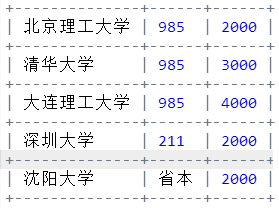data = [ ["北京理工大学", "985", 2000], \
["清华大学", "985", 3000], \
["大连理工大学", "985", 4000], \
["深圳大学", "211", 2000], \
["沈阳大学", "省本", 2000], \
]
from tabulate import tabulate
print(tabulate(data, tablefmt="grid"))

期末测验
*编程测试：
无空隙回声输出：获得用户输入，去掉其中全部空格，将其他字符按收入顺序打印输出
print(input().replace(" ",""))

文件关键行数：关键行指一个文件中包含的不重复行。关键行数指一个文件中包含的不重复行的数量。‪‬‪‬‪‬‪‬‪‬‮‬‪‬‫‬‪‬‪‬‪‬‪‬‪‬‮‬‭‬‫‬‪‬‪‬‪‬‪‬‪‬‮‬‫‬‫‬‪‬‪‬‪‬‪‬‪‬‮‬‫‬‮‬‪‬‪‬‪‬‪‬‪‬‮‬‫‬‫‬‪‬‪‬‪‬‪‬‪‬‮‬‫‬‮‬统计附件文件中关键行的数量
f = open("latex.log", "r", encoding="utf-8")
d = {}
for line in f:
d[line] = d.get(line, 0) + 1
print("共{}关键行".format(len(d)))

#参考答案：如果需要"去重"功能，请使用集合类型
f = open("latex.log")
s = set(ls)
print("共{}关键行".format(len(s)))

剩余两题与测验九重复，不重复记录
展开全文• ## Python内置函数

万次阅读 多人点赞 2019-05-10 15:10:36
如果iterable的所有元素不为0、’’、False或者iterable为空，all(iterable)返回True，否则返回False； 注意：空元组、空列表返回值为True，这里要特别注意。 all(['a', 'b', 'c', 'd']) # 列表list，元素都不为...Python内置函数
• 将中缀表达式转换后缀表达式 遵循以下步骤： (1) 初始化两个栈：运算符栈S1和储存中间结果的栈S2； (2) 从左至右扫描中缀表达式； (3) 遇到操作数时，将其压入S2； (4) 遇到运算符时，比较其与S1栈顶运算符的...
• 数据结构实习——算术表达式 一、实习题目及要求 1、题目：算术表达式 2、要求: （1）正确解释表达式； （2）符合四则运算规则： （3）先乘除、后加减； （4）从左到右运算； （5）先括号内，后括号外； （6...数据结构 c++
• 现在已经有很多开源可用的 java 表达式引擎,什么还需要 Avaitor 呢? Aviator的设计目标是轻量级和高性能,相比于Groovy、JRuby的笨重, Aviator非常小, 加上依赖包也才 537K,不算依赖包的话只有 70K; 当然, ...Aviator scala
• ## 前端面试题

万次阅读 多人点赞 2019-08-08 11:49:01前端面试题
• el表达式 验证运算 结果 \${empty param.username }${empty param.username }html import java
• 乍一看是一个恒等式，返回true也没错，但是要注意一点的是，赋值语句=左边必须是变量，如果是常量编译器会报错，如图：，但如果左边是变量之后就只是一个字符串表达式了，而不是boolean类型，所以也存在返回true和...Java
• ## 测试开发需要学习的知识结构

万次阅读 多人点赞 2018-04-12 10:40:58
2)a = flase, b = flase 3）条件覆盖：条件覆盖是指选择足够的测试用例，使得运行这些测试用例时，判定中每个条件的所有可能结果至少出现一次，但未必能覆盖全部分支 条件覆盖要检查每个符合谓词的子表达式值为真和...测试开发
• ## 测试开发笔记

万次阅读 多人点赞 2019-11-14 17:11:58
代码走查 测试过程 上一阶段下个阶段提供重点指导 用户参与的测试或用户反映回来的错误和问题下次测试的或测试补充的必备内容 第二章 测试过程 1.测试模型 H模型： H模型图 优点： 1 介入早 与开发并行 更早的...测试开发
• 本章介绍C#语言的基础知识，希望具有C语言的读者能够基本掌握C#语言，并以此基础，能够进一步学习用C#语言编写window应用程序和Web应用程序。当然仅靠一章的内容就完全掌握C#语言是可能的，如需进一步学习C#语言...c#
• 如果需要，非 Boolean 表达式也可以被转换 Boolean ，但是要遵循下列规则： 所有的对象都被当作 true。当且仅当字符串空时，该字符串被当作 false。null 和 undefined 被当作 false。当且仅当数字零时...js boolean
• 主要思路就是把式子转化成后缀表达式，然后再用后缀表达式的方法来计算，其中每个命题变元的情况是用没有剪枝的深搜枚举出来的 下面是代码 ps:第一版代码的条件语句计算出了点问题，已经改了，顺便加了个多组...
• 今天在论坛里面逛发现一个问题比较典型，我们在使用js的时候可以直接使用Eval得到某一个表达式，在C#里面可以吗？答案是肯定的，在.NET提供的丰富的类库里面这是可以实现的，但是没有js那么简单下面我将使用一个...
• 2、 已知a[n]整数数组，试写出实现下列运算的递归代码(C或C++代码均可)： 要求： a. 求数组中的最大整数； b. 求n个数的和； c. 利用堆栈类，将本题a和b的代码改成非递归的方式。 实验报告...
• 【简答题】尝试用颜色表达酸甜苦辣的感觉 !【填空题】Python语句print("hello" "world")的执行结果是【简答题】...【简答题】下列Python语句的运行结果是 x=False; y=True; z=False if x or y a...
• 以下表达式的运行结果是： [1&amp;amp;amp;lt;2&amp;amp;amp;lt;3,3&amp;amp;amp;lt;2&amp;amp;amp;lt;1] A.[true,true] B.[true,false] C.报错 D.其他 答案选： A 问题：什么js 1&amp;amp;...
• 【实验目的】 使学生深入了解栈的特性，以便在实际...利用教科书表3.1给出的算符优先关系，实现对算术四则混合运算表达式的求，并仿照教科书的例3-1演示在求中运算符栈、运算数栈、输入字符和主要操作的变化过程。数据结构 c++
• ## Java笔试面试题整理第八波

万次阅读 多人点赞 2016-06-14 11:07:41
同样List也能转化List。 3、变量初始化问题 对于成员变量： 对于 非final修饰 的类的 成员变量（包括static和非static） ，如果开发者没有给其赋初值，在编译时，JVM自动会给非final修饰的成员...Java面试题 Java笔试题 泛型问题 变量初始化
• 1、 表达式 ‘abc’ in ‘abcdefg’ 的值为_True_，表达式 ‘abc’ in [‘abcdefg’] 的值为___False___。 2、 Python语句’’.join(list(‘hello world!’))执行的结果是’hello world!’。 3、 已知列表对象x = ...字符串 python
• ## 【MATLAB】MATLAB的基础知识

千次阅读 多人点赞 2017-04-12 11:52:00
语句组 end 其中m循环起始，n循环终止，p步长。 例 1-6. 我们用 for 语句来实现求和运算 s = 1+3+5+7+…+99，对应的MATLAB命令如下： s = 0; for i = 1:2:99 s = s+I; end s ...matlab
• 布尔表达式和正则表达式Example 1: Simplify the given Boolean Expression ... 示例1：将给定的布尔表达式简化最小编号。 变量或文字。 (A+B). (A+B) (A + B)。 (A + B ) ABC + AB + ABC ABC + A B + AB C Answ...java webview lambda
• 上周末参照书本写了个“计算器”的程序，其中最令我费解的就是“前序表达式”、“后续表达式”，好像记得老师在上课的时候讲过，估计当时也没听懂，看的稀里糊涂的，不过现在大概明白了…… 　在此仅做以笔记。 ...算法
• ## WPF开发教程

万次阅读 多人点赞 2019-07-02 23:13:20
因为对象可以有数十个（如果达到上百个）属性，并且大部分处于其默认状态（被继承、由样式设置等），所以并非对象的每个实例都需要具有在该对象上定义的每个属性的完全权重。 属性系统的最后一个新功能是附加...WPF
• 这里MDN已经有专门的的解释，却别与布尔true，对于表达式返回真值统称Truthy. Truthy（真值） 在 JavaScript 中，truthy（真值）指的是在布尔上下文中，转换后的值为真的。 所有都是真值，除非它们被......